Function Repository Resource:

# CombinatorGlocalMultiwaySystem

Simulate the evaluation of a combinator expression as a "glocal" (hybrid of global and local) multiway system

Contributed by: Jonathan Gorard
 ResourceFunction["CombinatorGlocalMultiwaySystem"][rules,init,n] generates the results of n steps in the glocal multiway evaluation of a combinator expression with the specified rules, starting from initial conditions init. ResourceFunction["CombinatorGlocalMultiwaySystem"][rules→sel,init,n] uses the function sel to select which of the events obtained at each step to include in the evaluation.

## Details and Options

Glocal multiway systems combine the global events (and the corresponding causal structure) of ordinary multiway systems with the individual token philosophy of local multiway systems. A single event vertex in the evolution causal graph (or token event graph) will, in general, have many incoming and outgoing evolution edges, corresponding to the fact that several tokens must be assembled together in order to reconstruct a single global input or output state for the event.
Argument and option patterns for ResourceFunction["CombinatorGlocalMultiwaySystem"] match those of the resource function MultiwayCombinator (and, to a lesser extent, the resource function MultiwaySystem).
Rule and state specifications for ResourceFunction["CombinatorGlocalMultiwaySystem"] are rules and expressions of some combinatory calculus.
ResourceFunction["CombinatorGlocalMultiwaySystem"] accepts both individual rules of combinatory calculus and lists of rules, and likewise for initial conditions. ResourceFunction["CombinatorGlocalMultiwaySystem"][rules,init,n] is interpreted as ResourceFunction["CombinatorGlocalMultiwaySystem"][rules,{init},n] etc.
The event selection function sel in ResourceFunction["CombinatorGlocalMultiwaySystem"][rulessel,] can have the following special forms:
 "Sequential" applies the first possible replacement (sequential substitution system) "Random" applies a random replacement {"Random",n} applies n randomly chosen replacements
Duplicated tokens are displayed separately at each time step by default, although this can be overridden by setting "DeduplicateTokens"True.
Events are represented in the form {rule,input,rest}, where rule is the rule used in the updating event, input is the part of the state to which the rule is applied and rest is the remainder of the state. For substitution systems, rest is given in the form {prefix,suffix}.
Options for ResourceFunction["CombinatorGlocalMultiwaySystem"] include:
 "DeduplicateTokens" False whether to merge all instances of equivalent tokens that appear at each time step "VertexRendering" True whether to use special rendering for state/token and event vertices "IncludeInitializationEvents" False whether to include pseudoevents that set up initial conditions "StateRenderingFunction" Automatic how to label states/tokens that appear in the evolution causal graph/token event graph "EventRenderingFunction" Automatic how to label events that appear in the evolution causal graph/token event graph
All of the standard options for the Graph function can also be applied to ResourceFunction["CombinatorGlocalMultiwaySystem"].
Possible settings for "StateRenderingFunction" and "EventRenderingFunction" include:
 Automatic make a label from the name of the vertex Inherited use the explicit vertex name as the label None use no label for the vertex "string" use a shape from the VertexShapeFunction collection func apply the function func to the name of the vertex

## Examples

### Basic Examples (3)

Generate evolution causal graphs (token event graphs) for two simple glocal multiway combinator evaluations for the fixed-point combinator (i.e. the Y combinator):

 In:=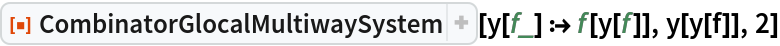Out=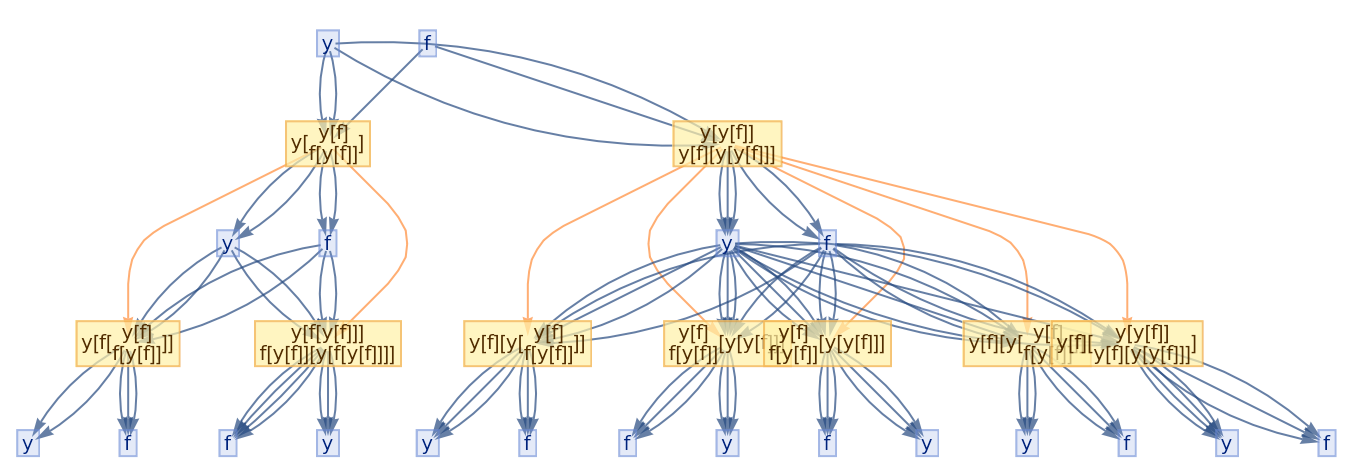In:=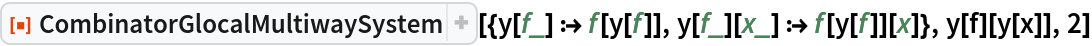Out=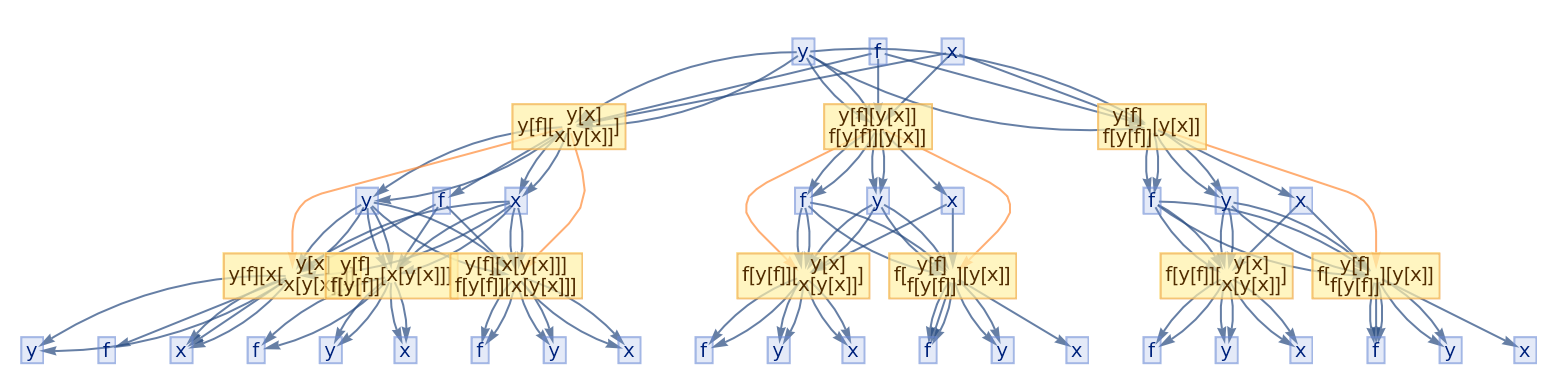Show just the structure of the graphs, without labels:

 In:=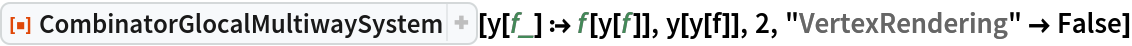Out=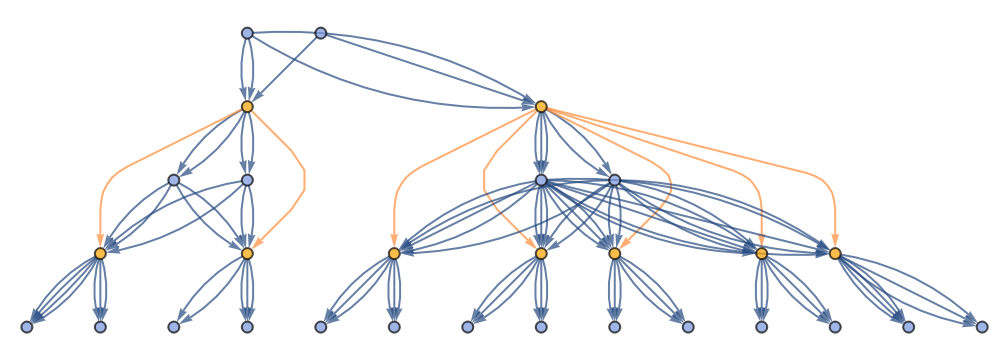In:=Out=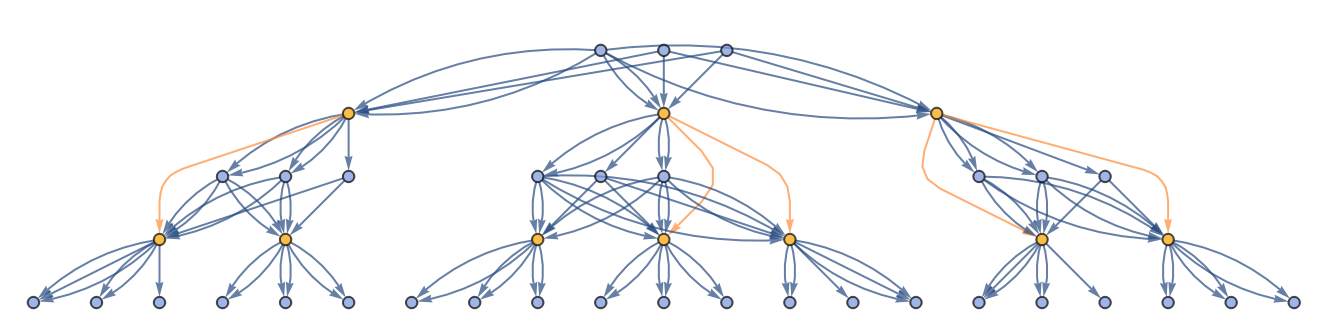Generate an evolution causal graph (token event graph) for a more complicated glocal multiway combinator evaluation for the S-K calculus:

 In:=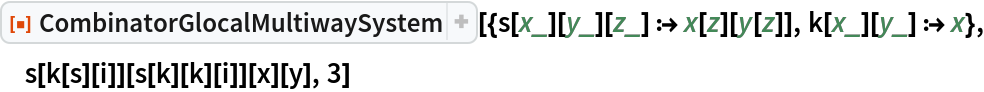Out=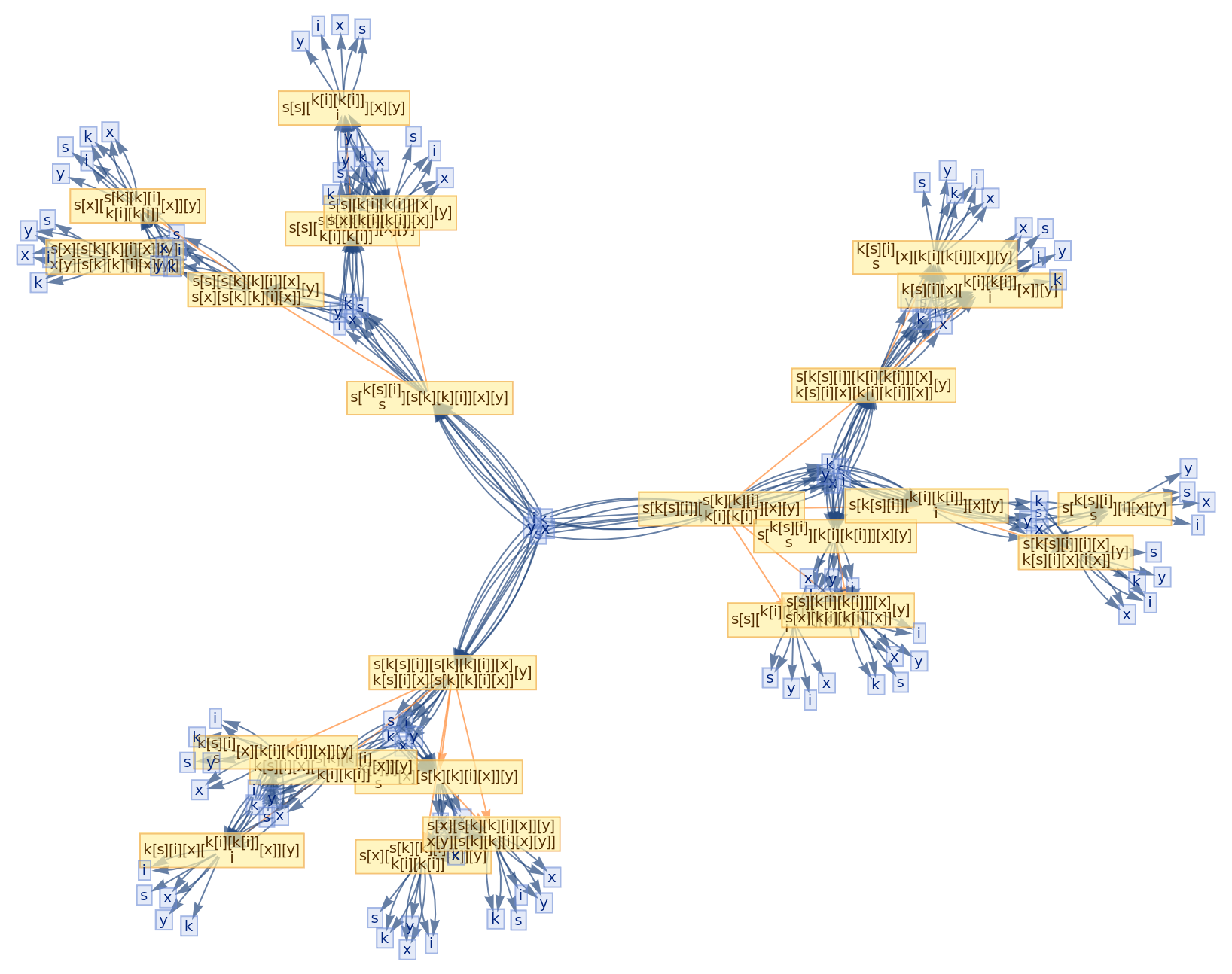Show just the structure of the graph, without labels:

 In:=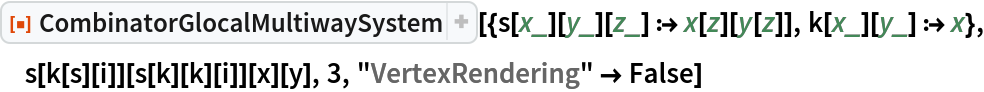Out=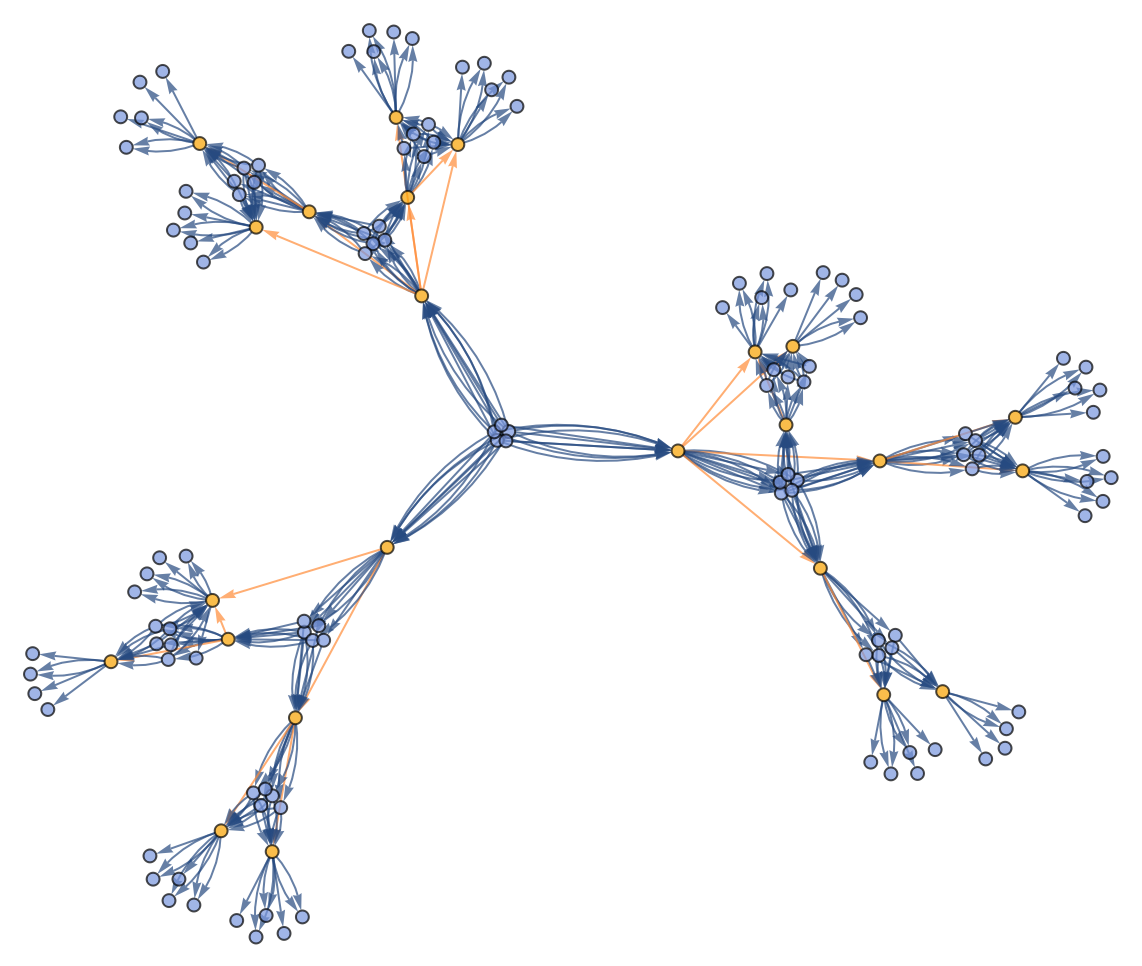Merge all instances of equivalent tokens that appear at each time step:

 In:=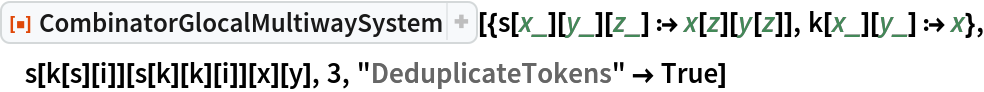Out=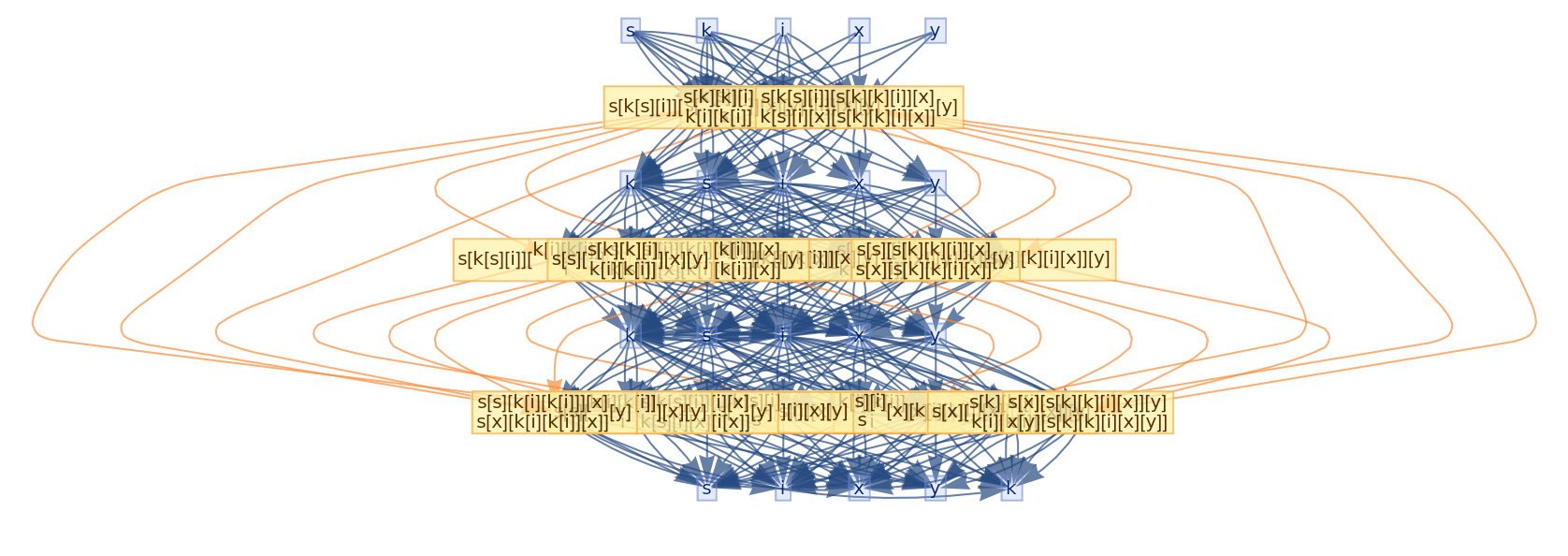Run the system for more steps:

 In:=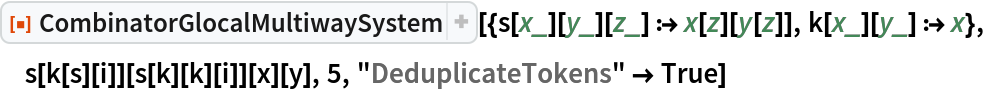Out=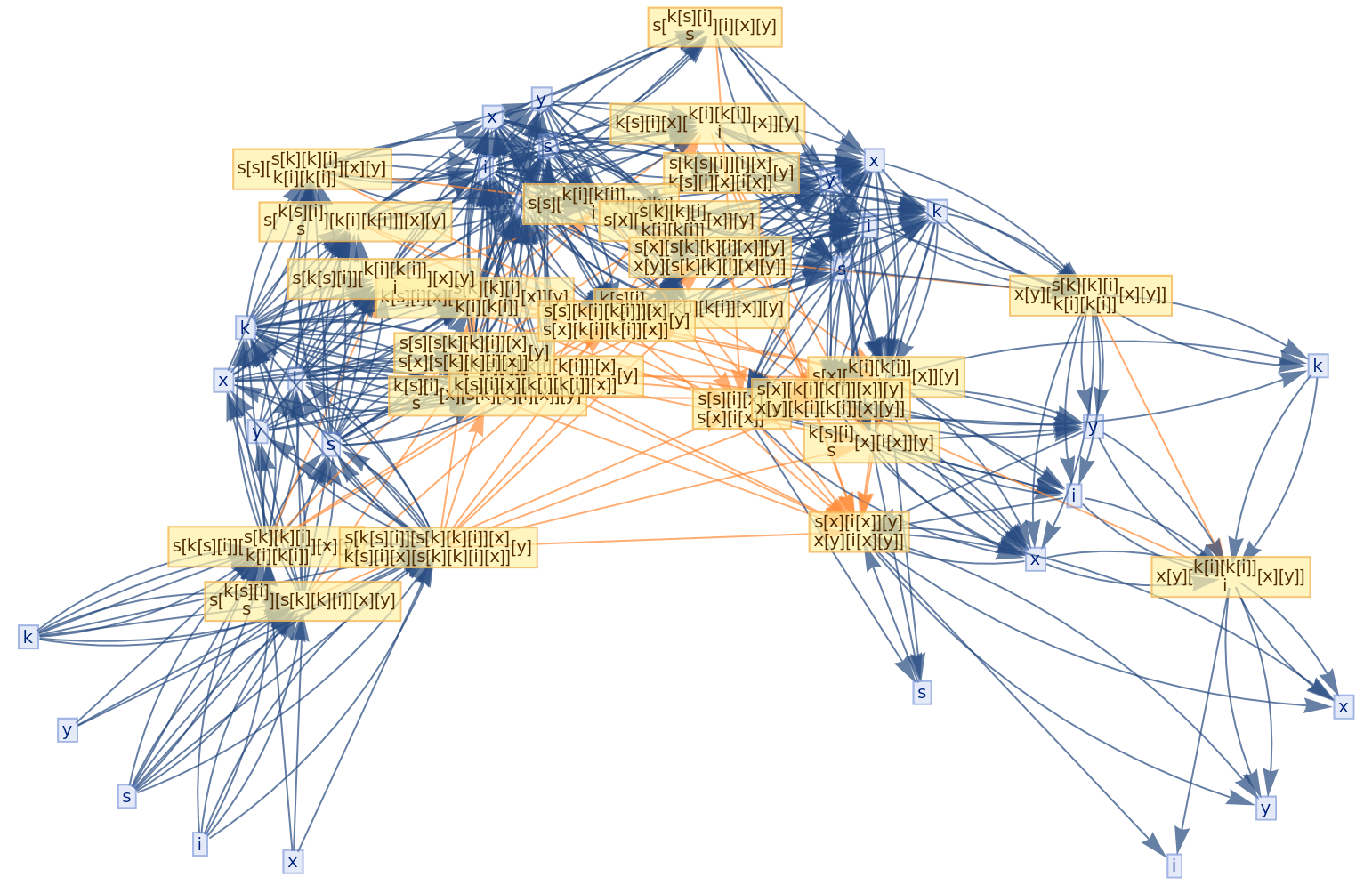Show just the structure of the graphs, without labels:

 In:=Out=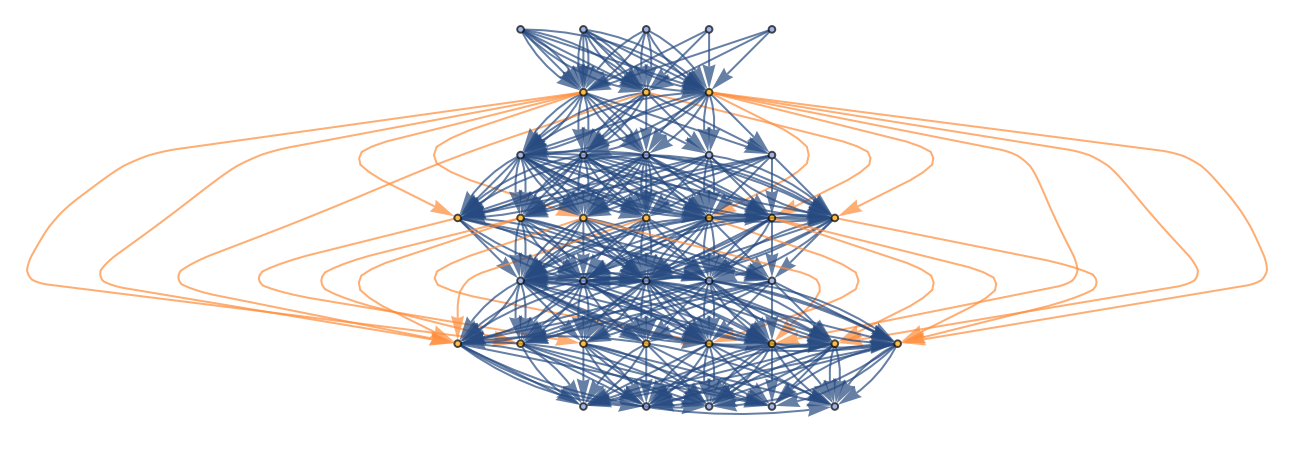In:=Out=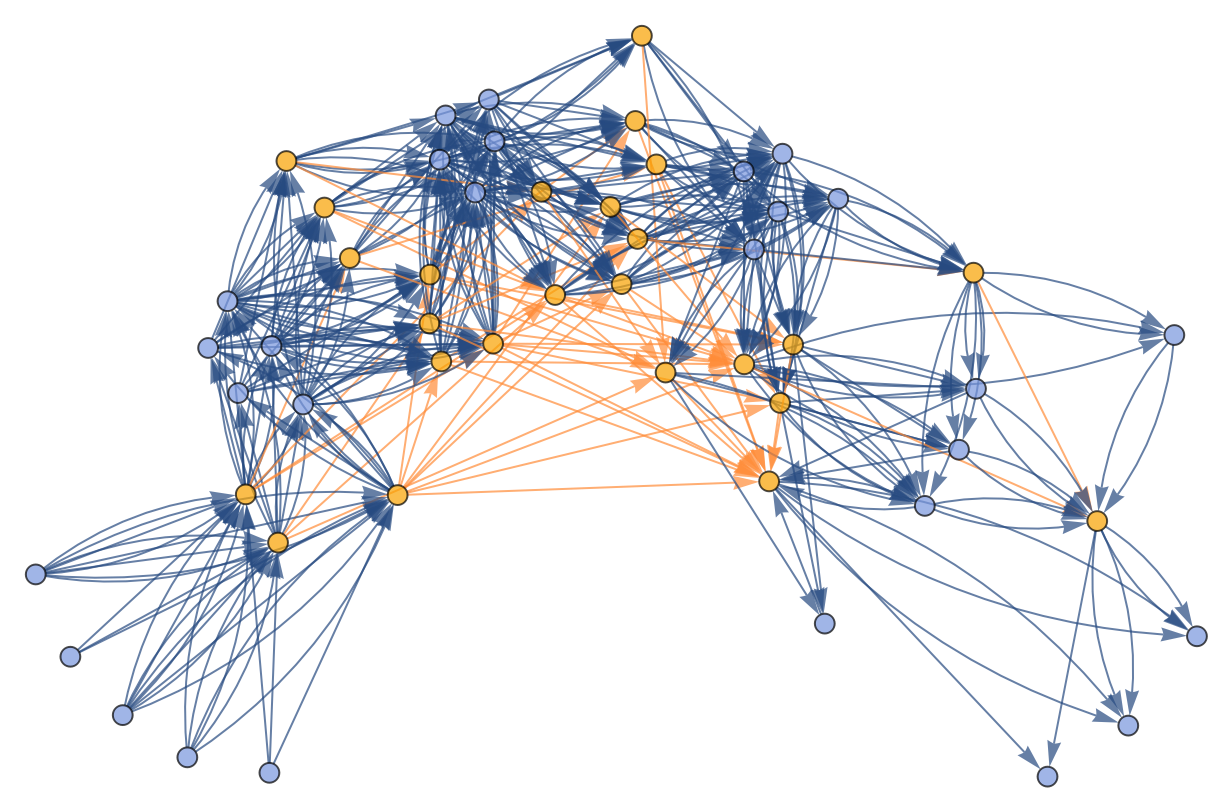Specify an event selection function that picks only up to two events at each step:

 In:=Out=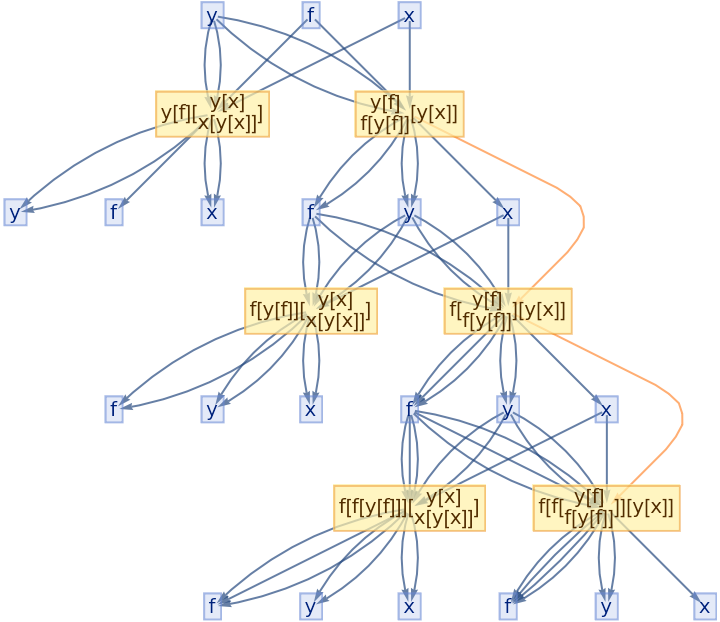### Scope (7)

#### Combinatory calculi (2)

CombinatorGlocalMultiwaySystem supports all forms of combinatory calculi, including the single-variable fixed-point (Y) combinator:

 In:=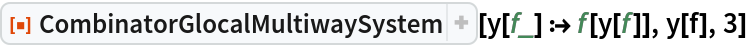Out=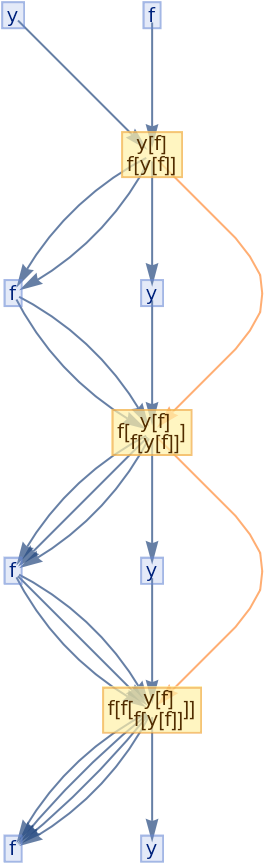The two-variable fixed-point (Y) combinator:

 In:=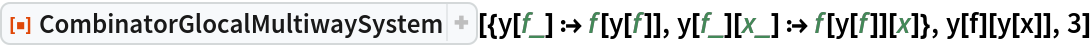Out=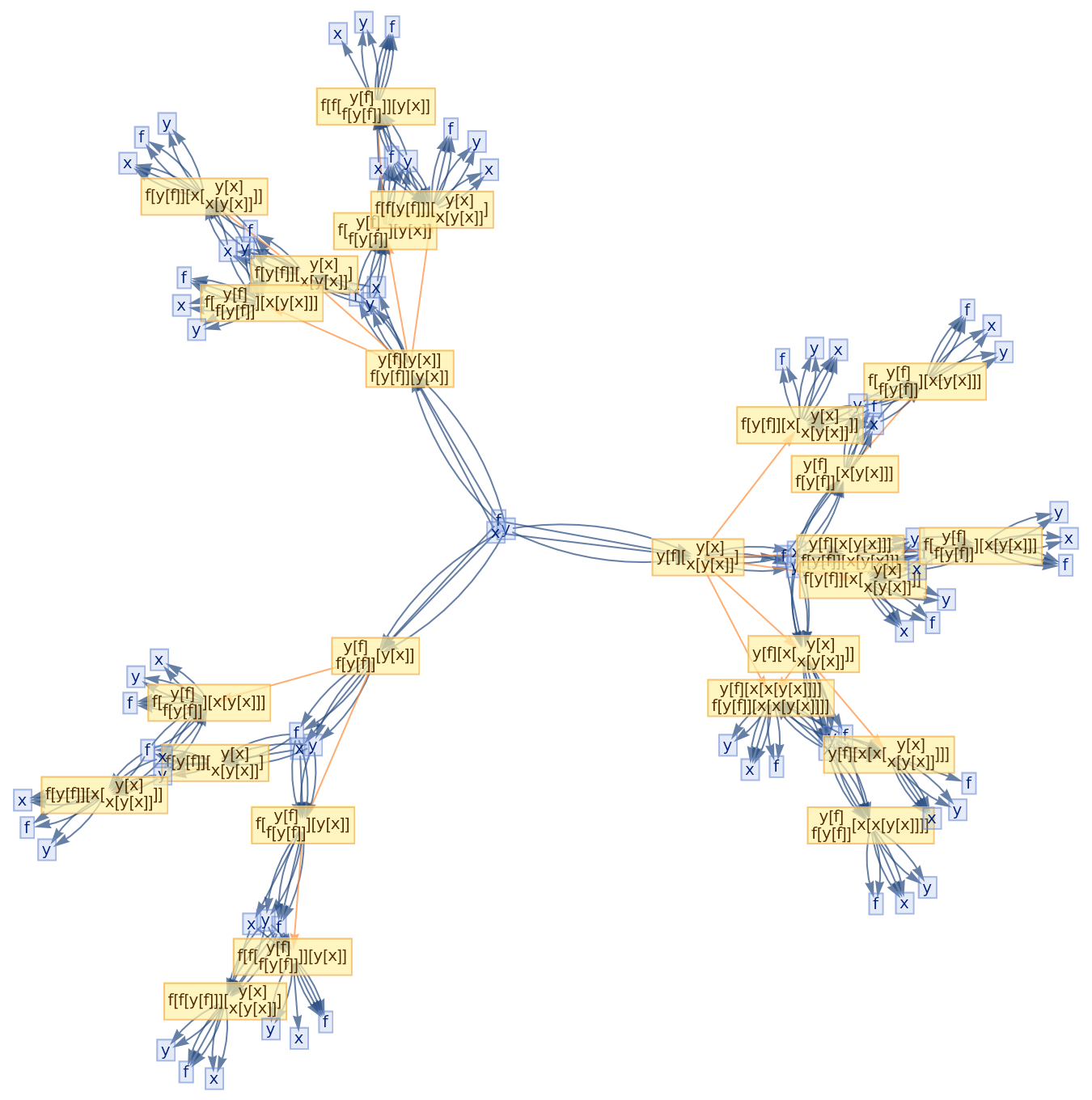The S-K calculus:

 In:=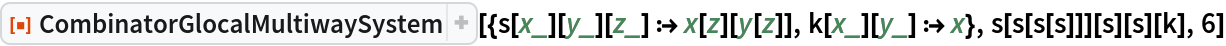Out=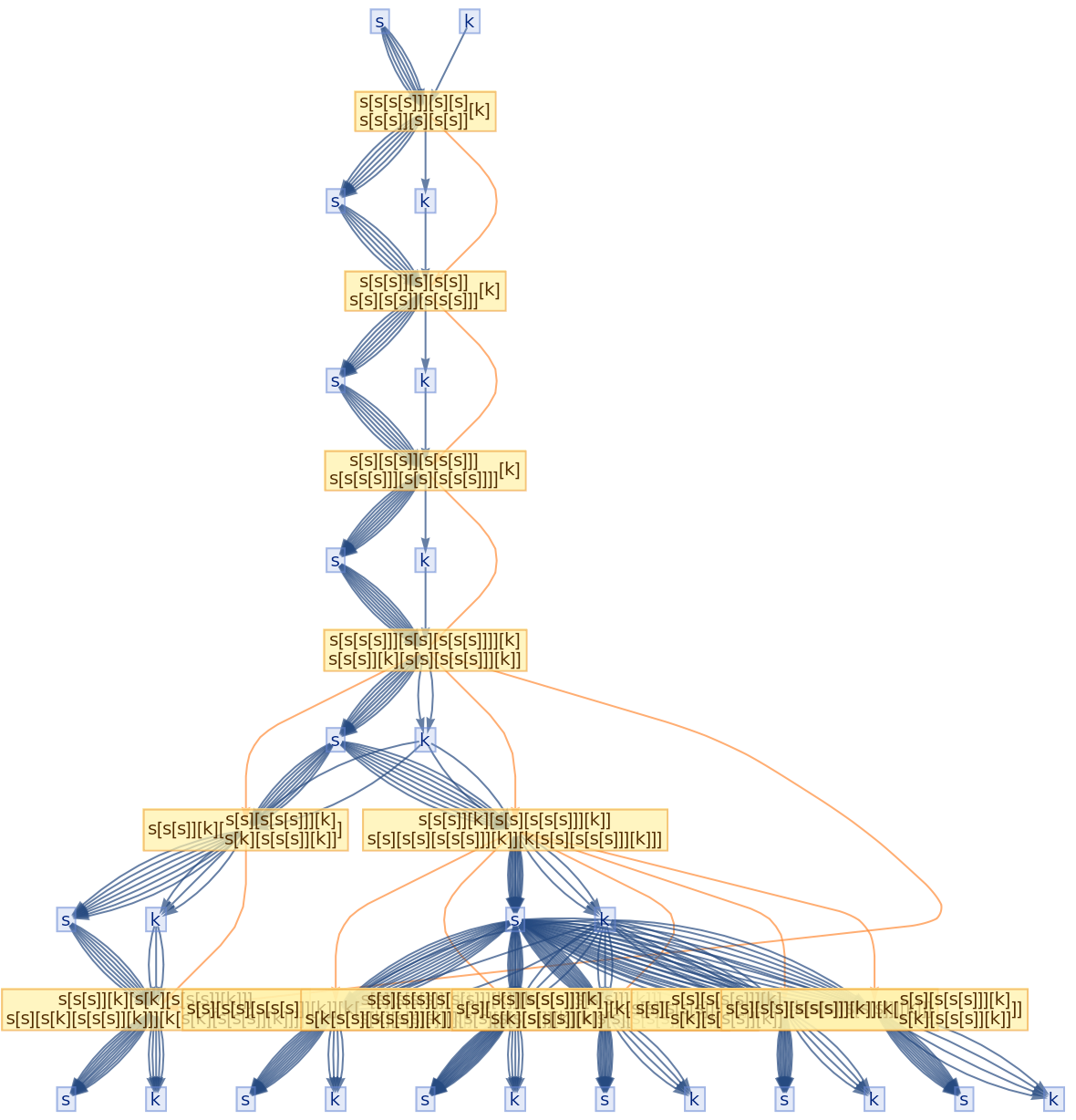The S-K-I calculus:

 In:=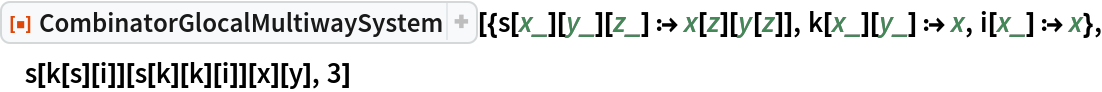Out=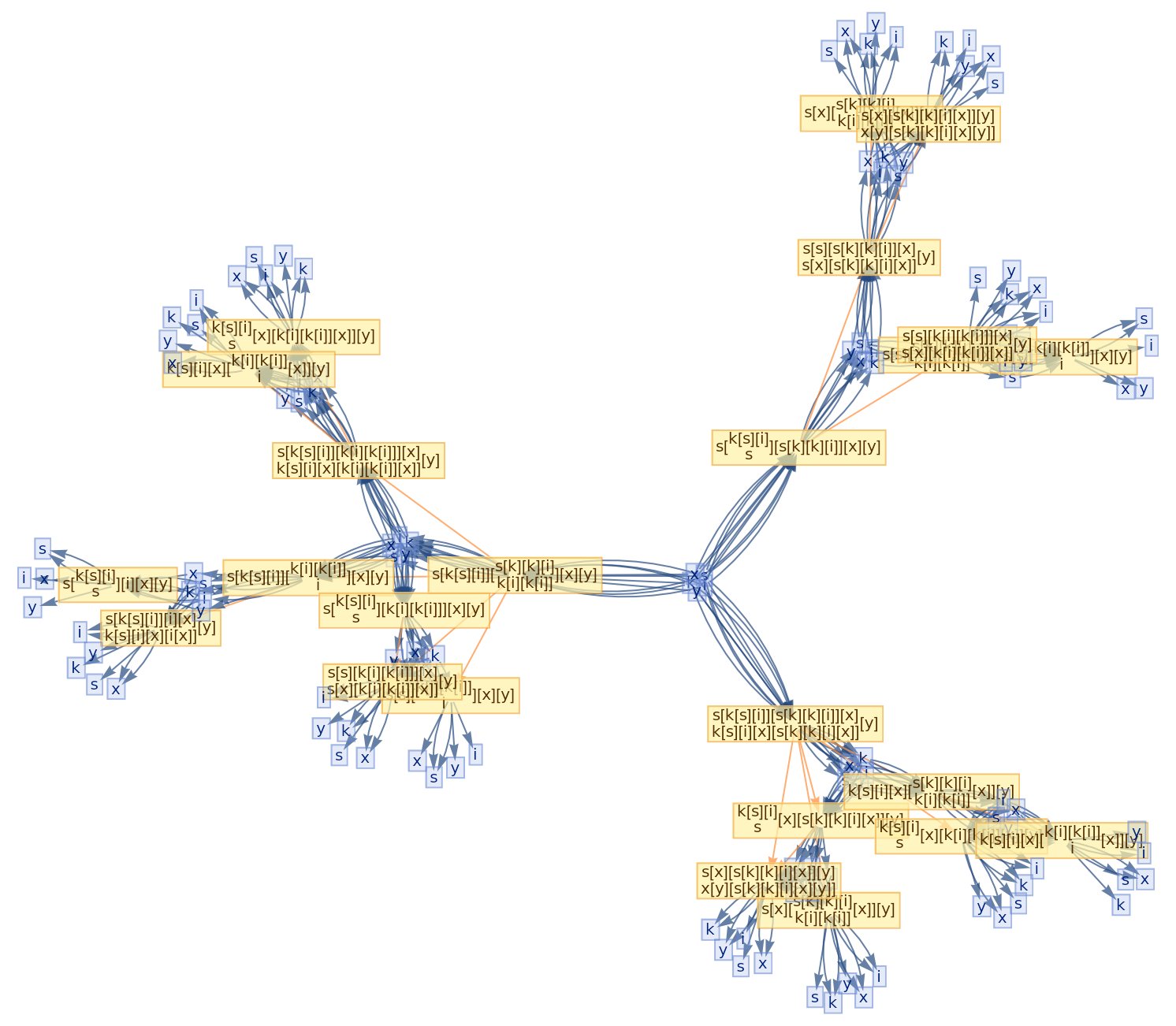Schönfinkel's B and C combinators:

 In:=Out=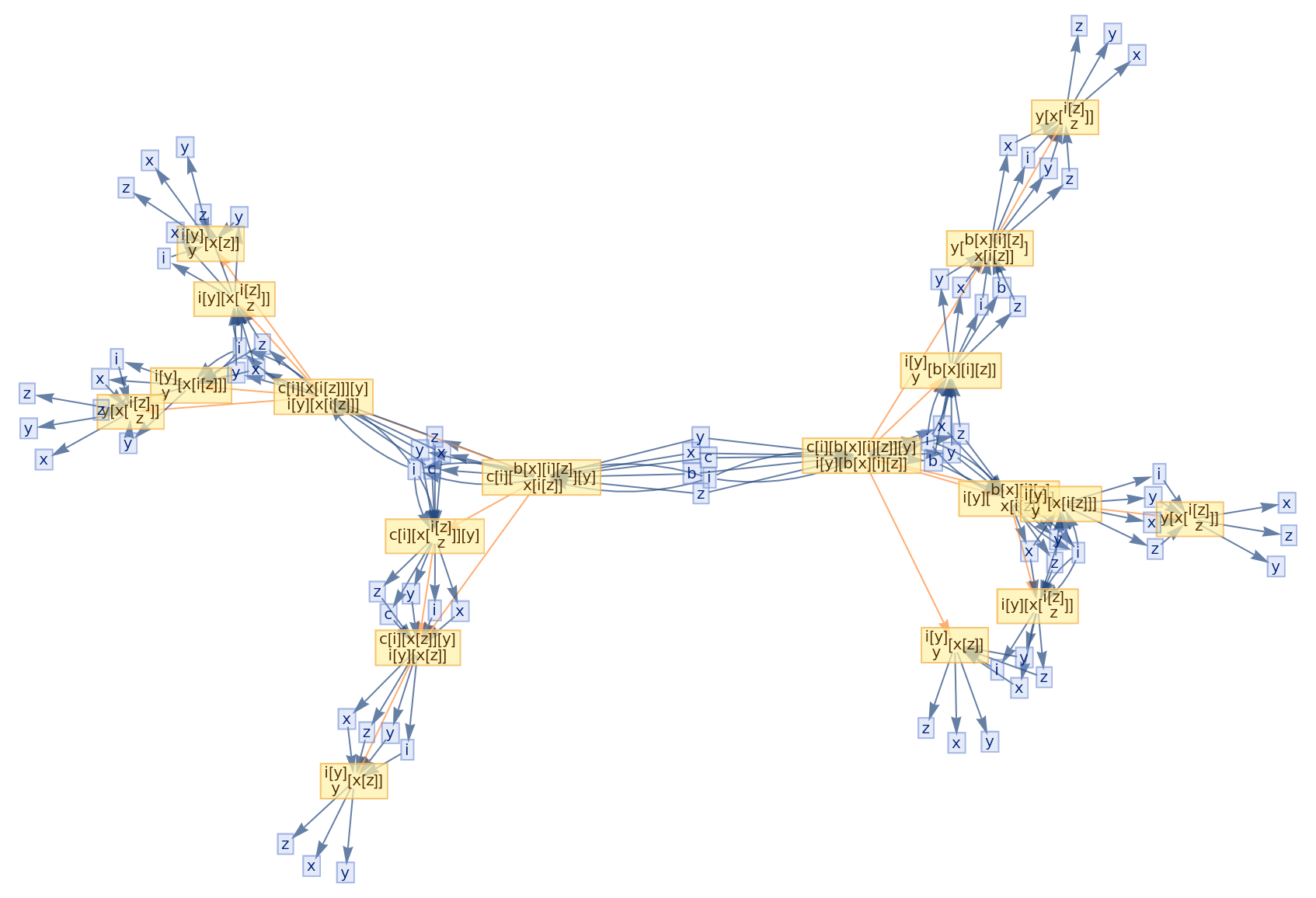Due to the undecidability of the S-K-I combinatory calculus, the following evaluation does not terminate at a normal form:

 In:=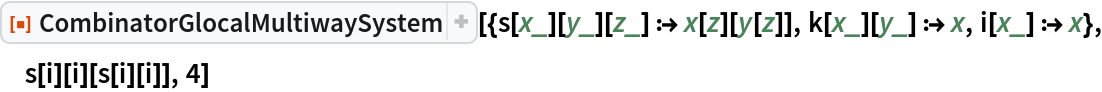Out=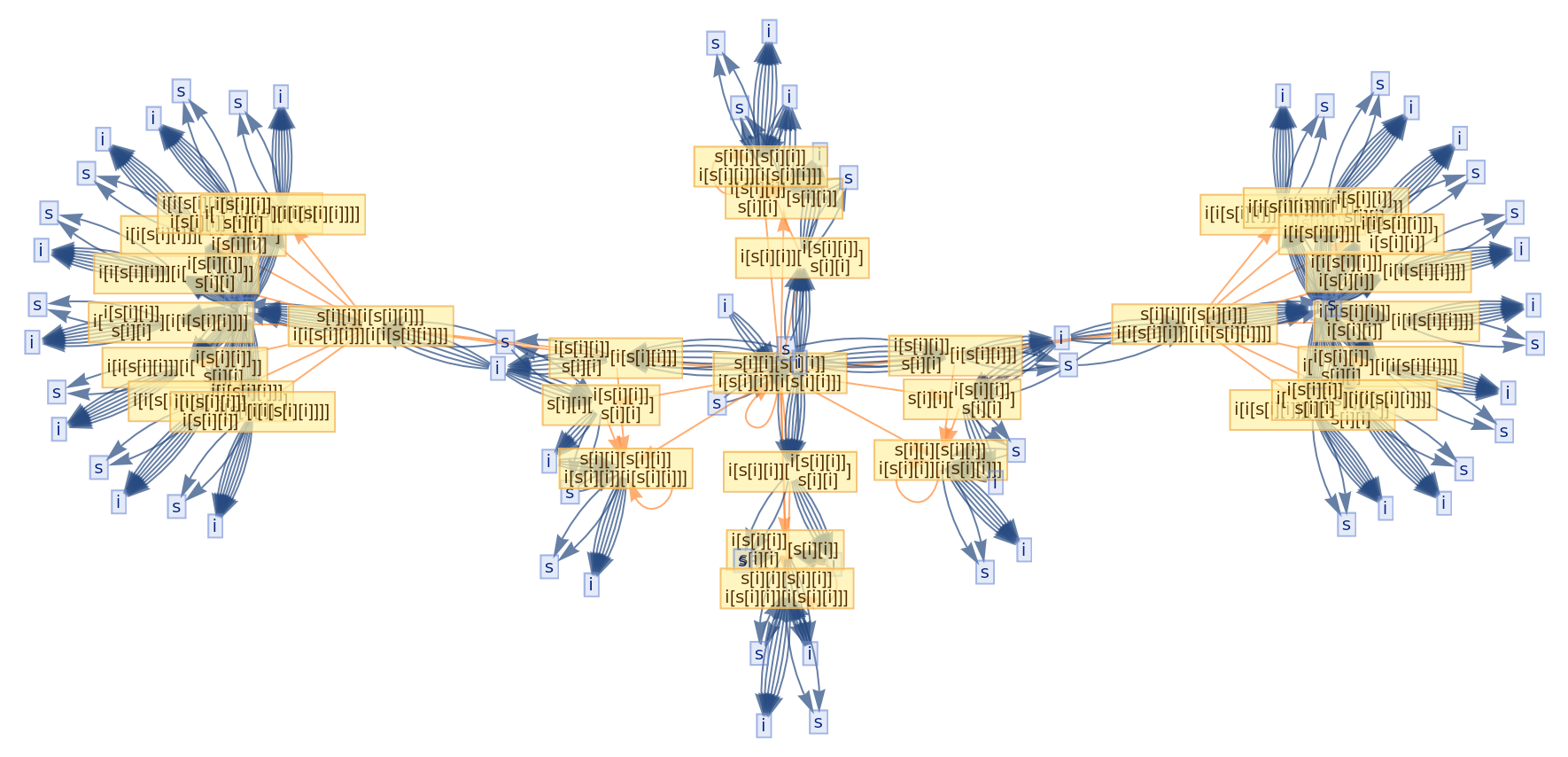#### Rules and initial conditions (2)

CombinatorGlocalMultiwaySystem accepts both individual combinator rules and lists of combinator rules:

 In:=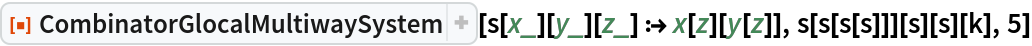Out=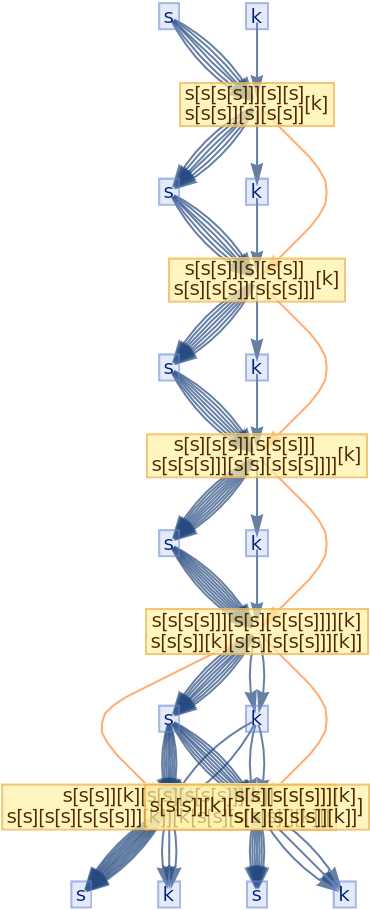In:=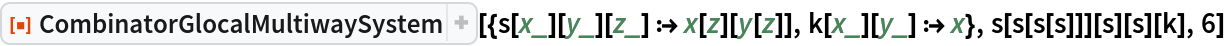Out=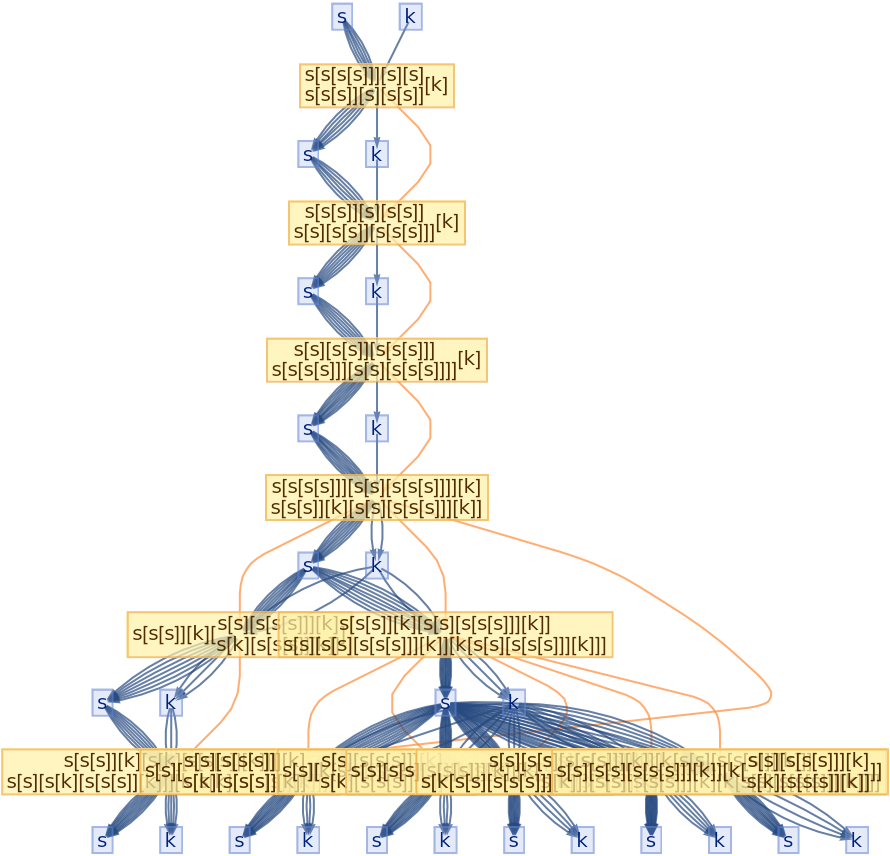CombinatorGlocalMultiwaySystem accepts both individual initial conditions and lists of initial conditions:

 In:=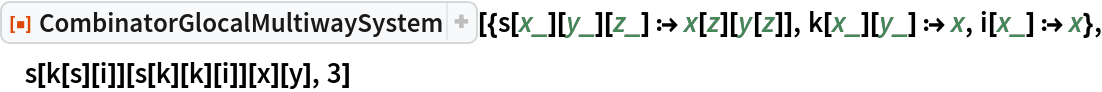Out=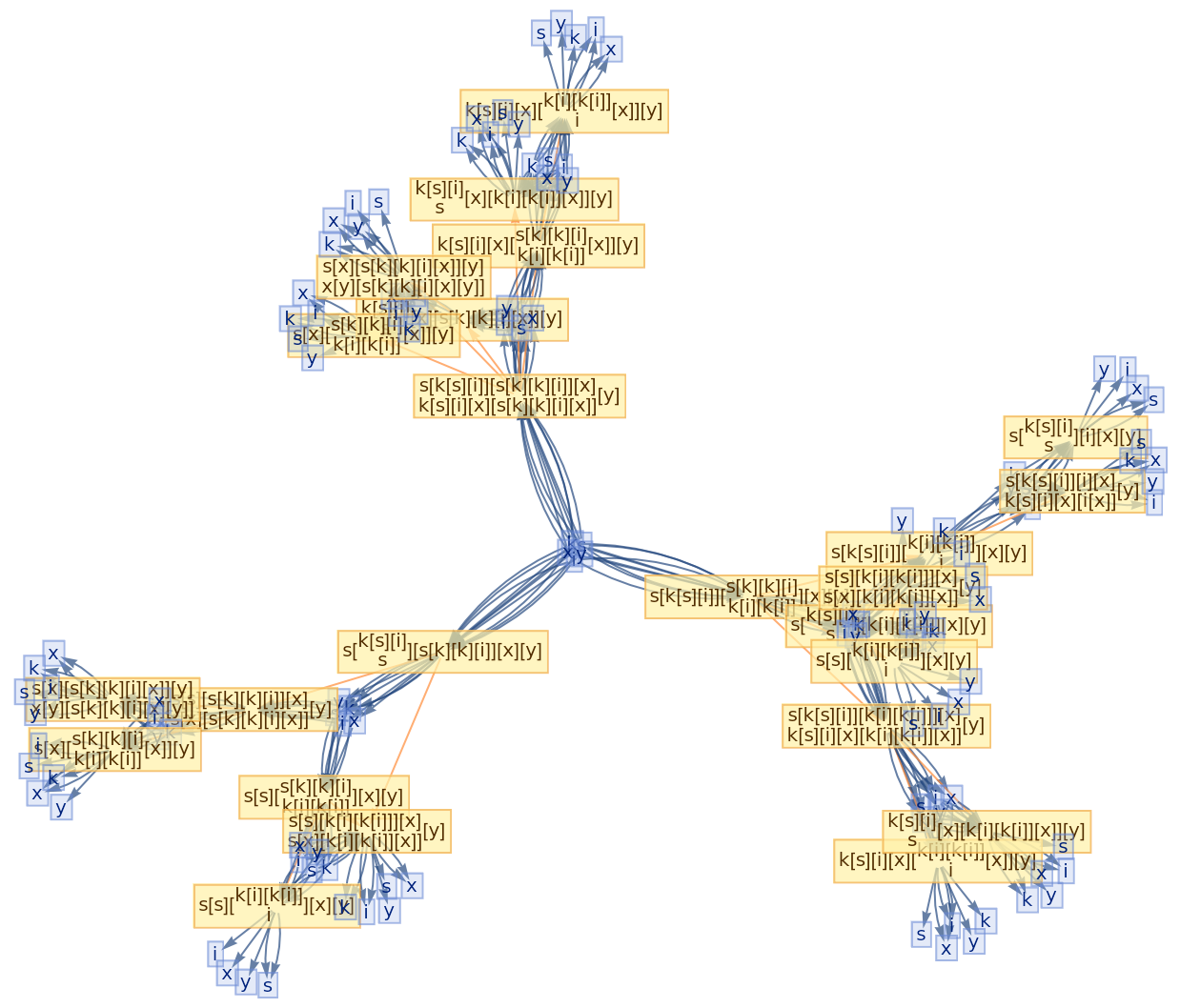In:=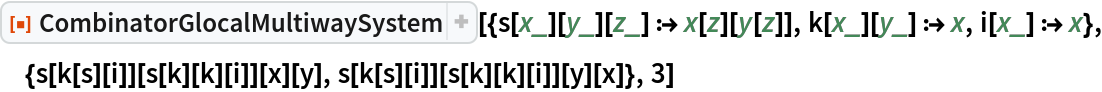Out=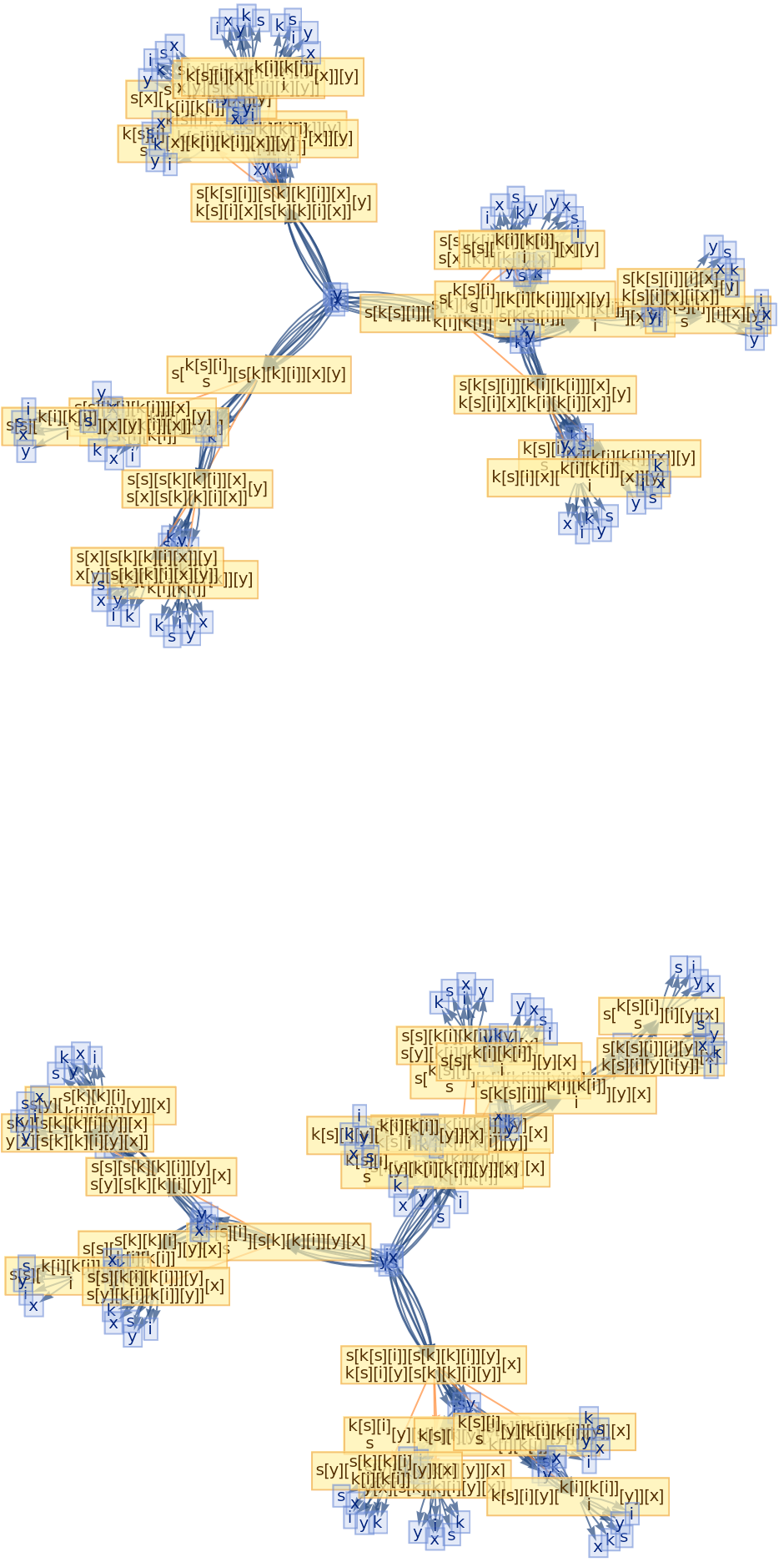#### Event selection functions (3)

Apply only the first possible event at each step:

 In:=Out=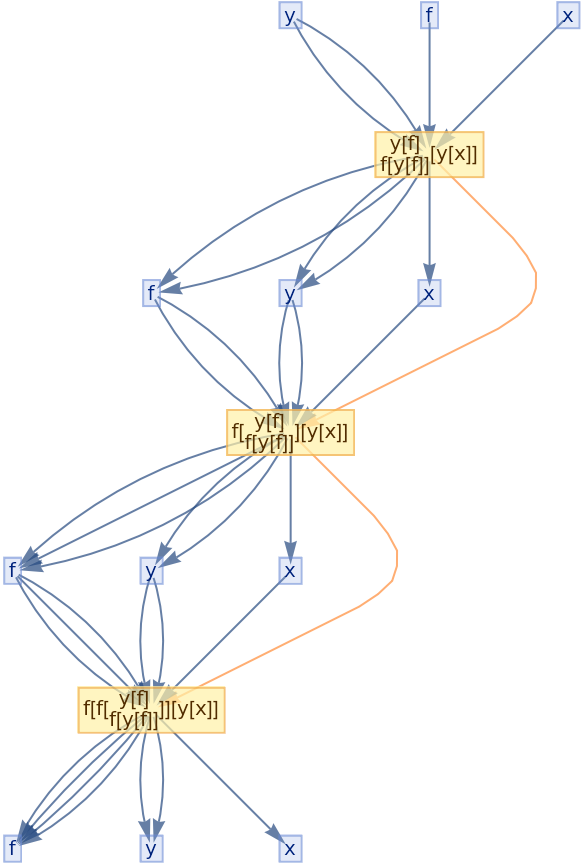Apply the first and last possible events at each step:

 In:=Out=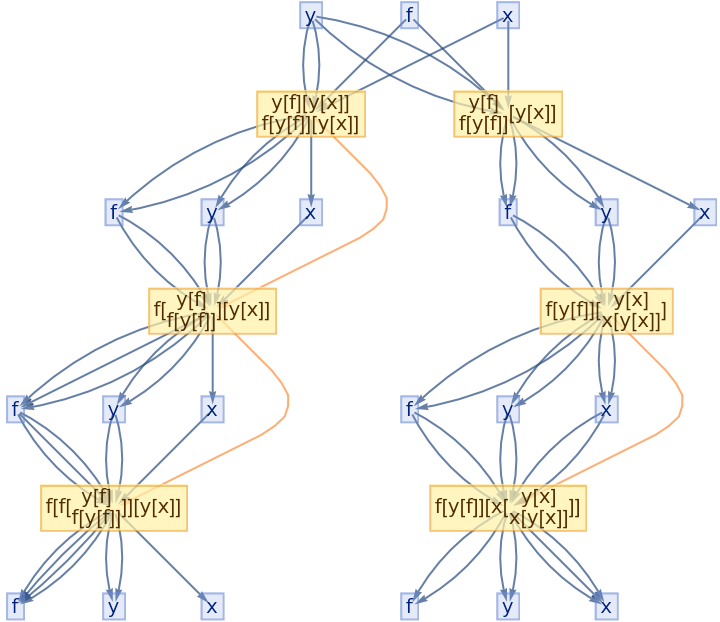Compare this to the full evolution causal graph (token event graph):

 In:=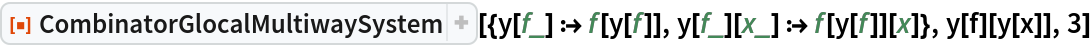Out=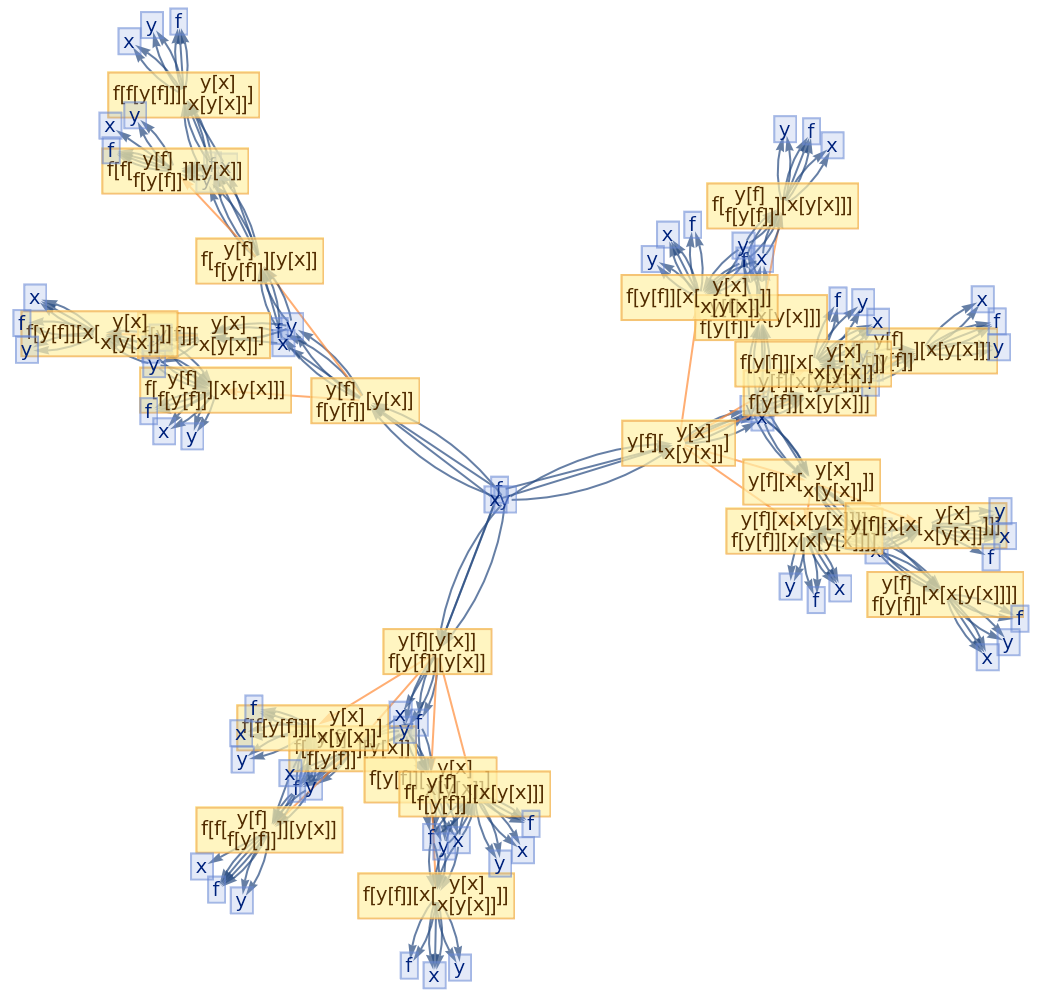### Options (19)

#### Token deduplication (2)

By default, equivalent tokens remain unmerged at each time step:

 In:=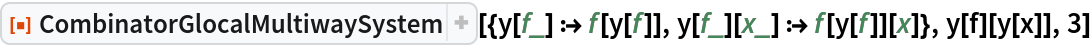Out=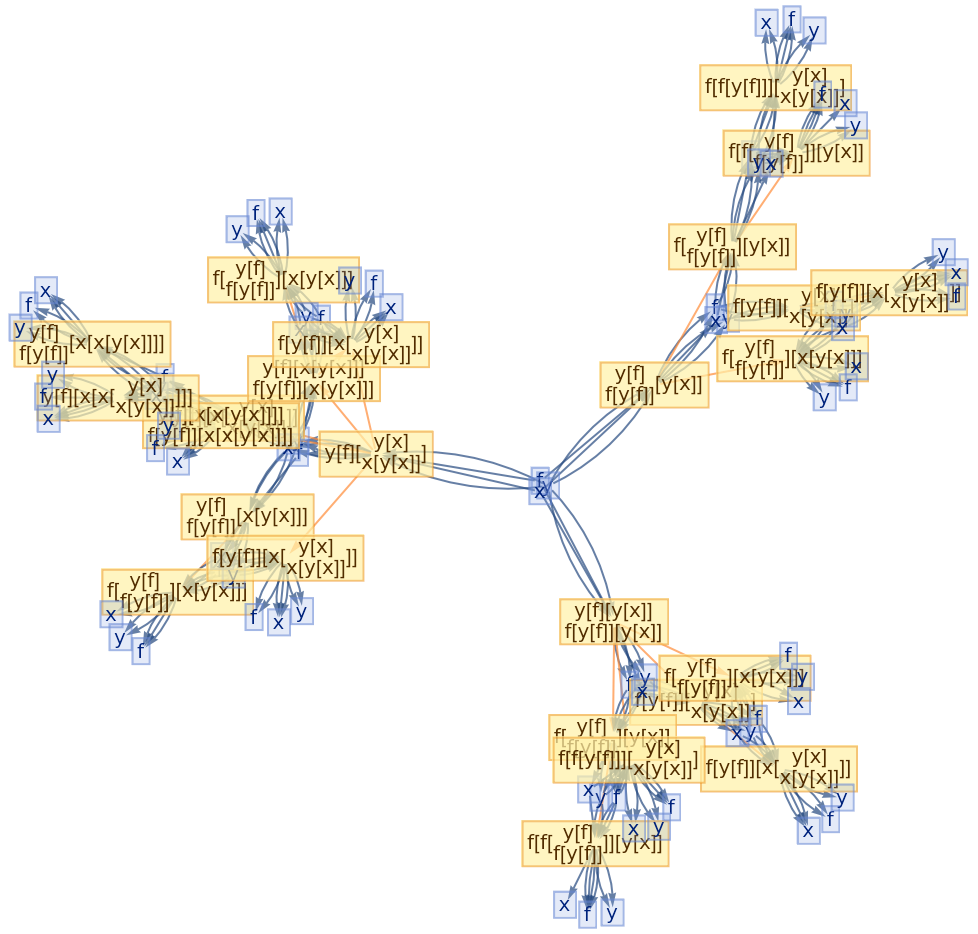Merging of equivalent tokens at each time step can be enforced using the option "DeduplicateTokens":

 In:=Out=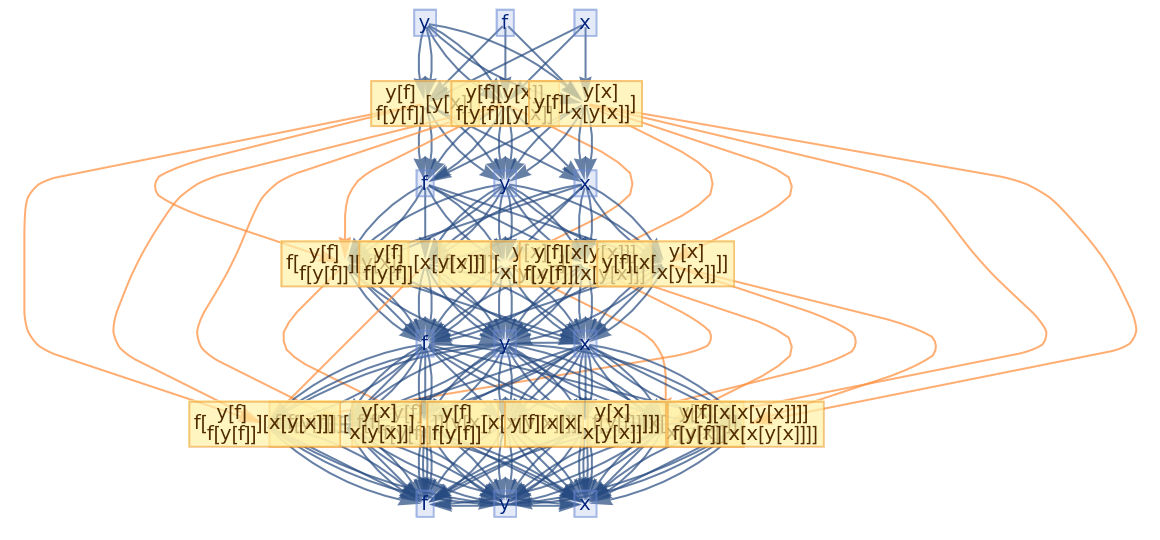#### Vertex rendering (2)

By default, state/token vertices and event vertices use special rendering (inherited from the MultiwayCombinator resource function):

 In:=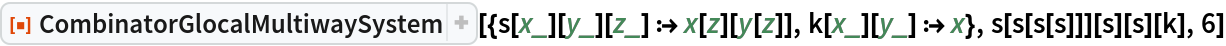Out=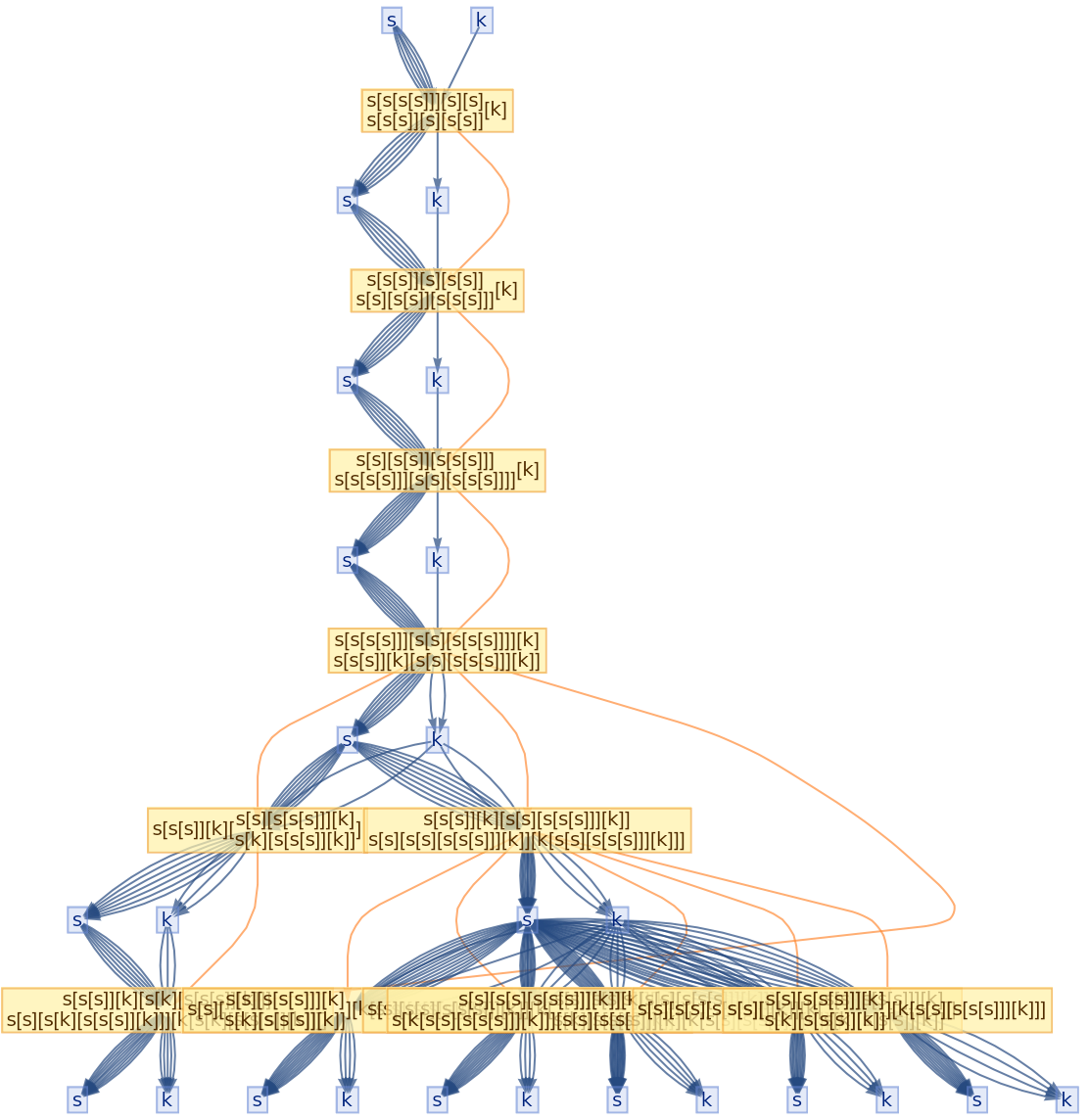This rendering can be disabled using the option "VertexRendering":

 In:=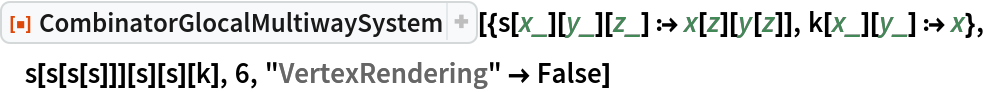Out=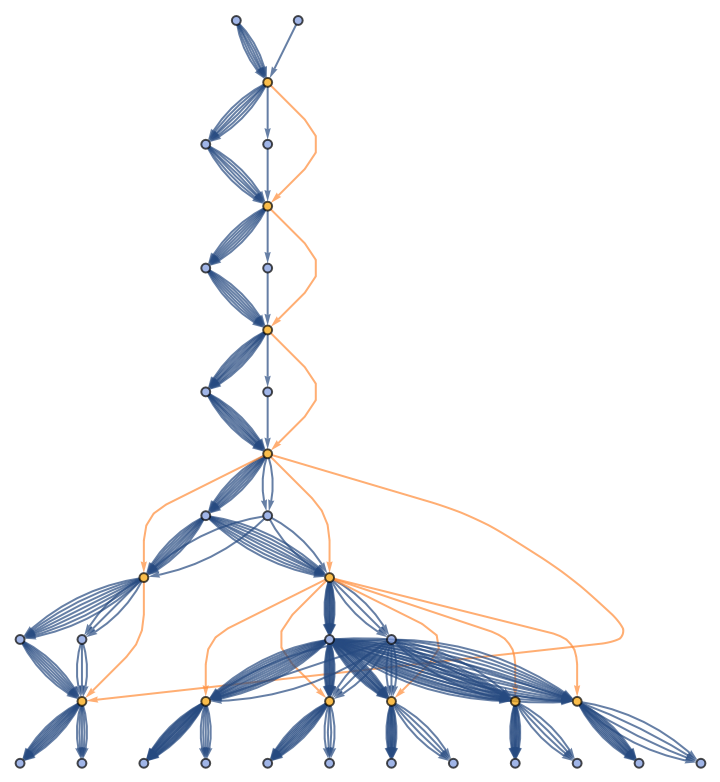#### State/Token vertex rendering (4)

By default, states/tokens are labeled by their contents:

 In:=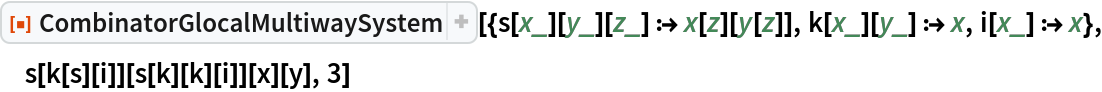Out=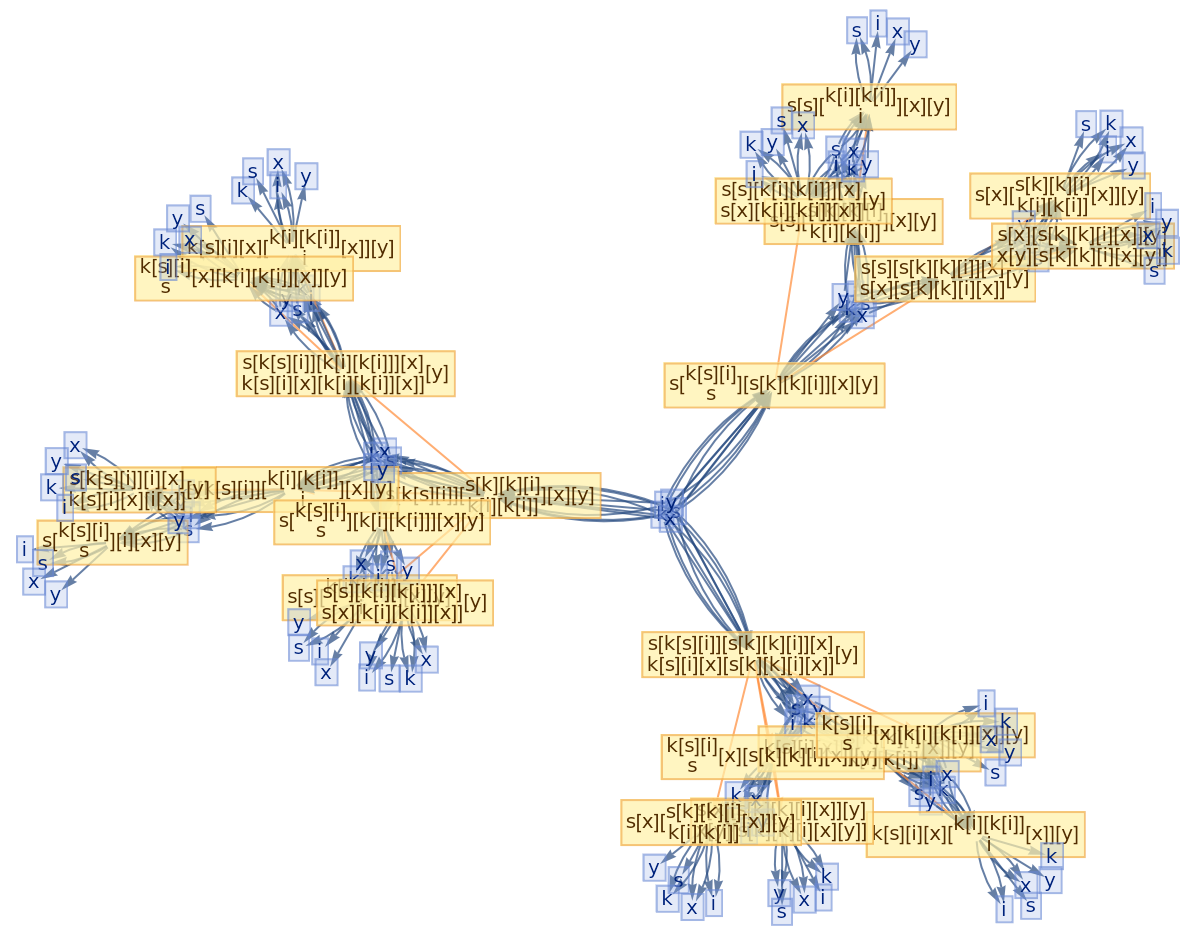Use no labeling for states/tokens:

 In:=Out=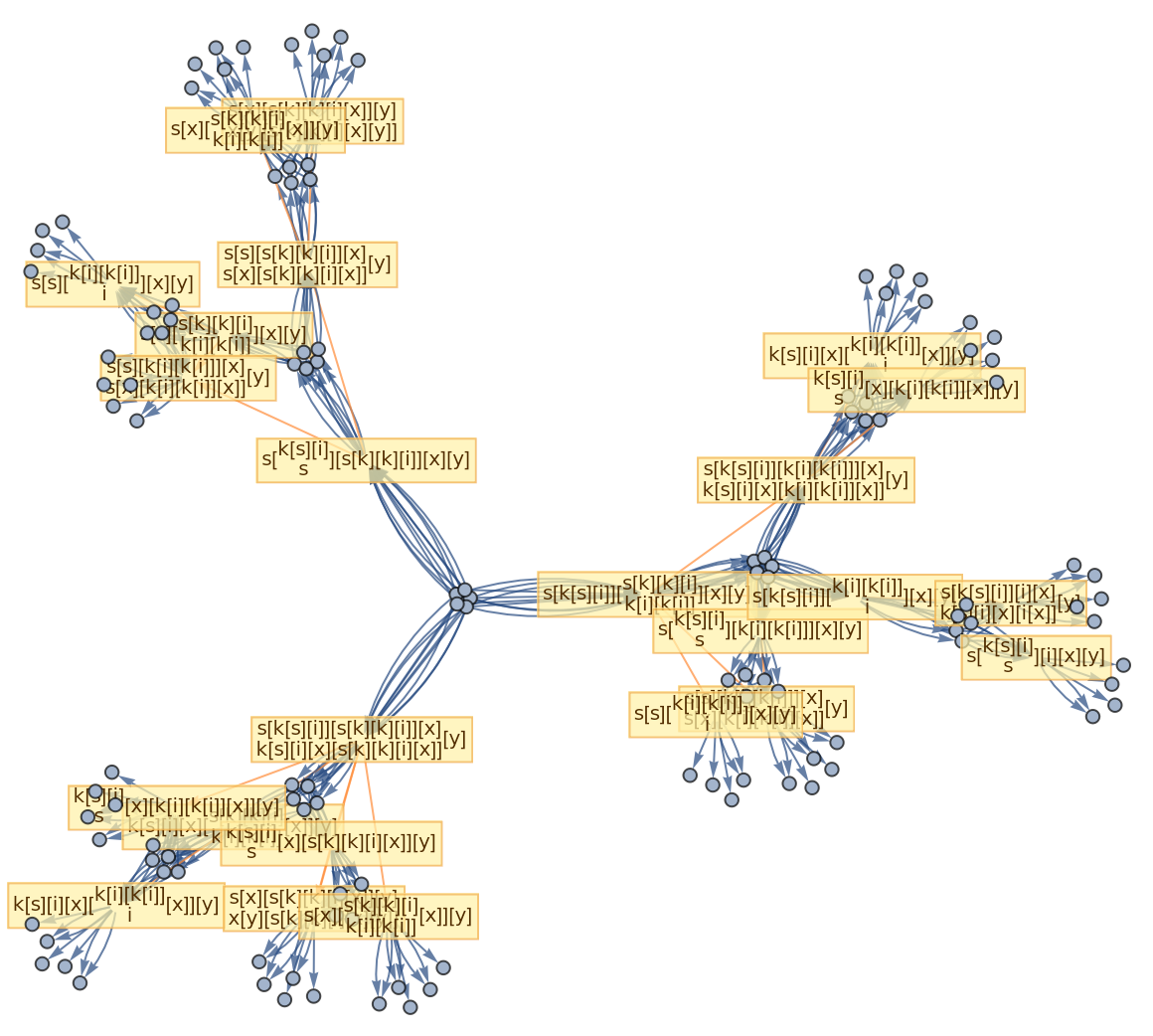Use raw state/token names as vertex labels:

 In:=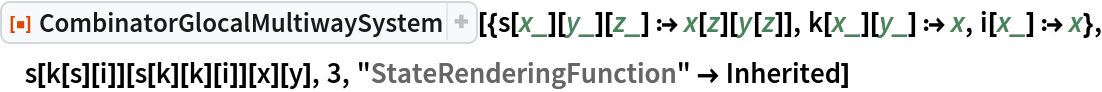Out=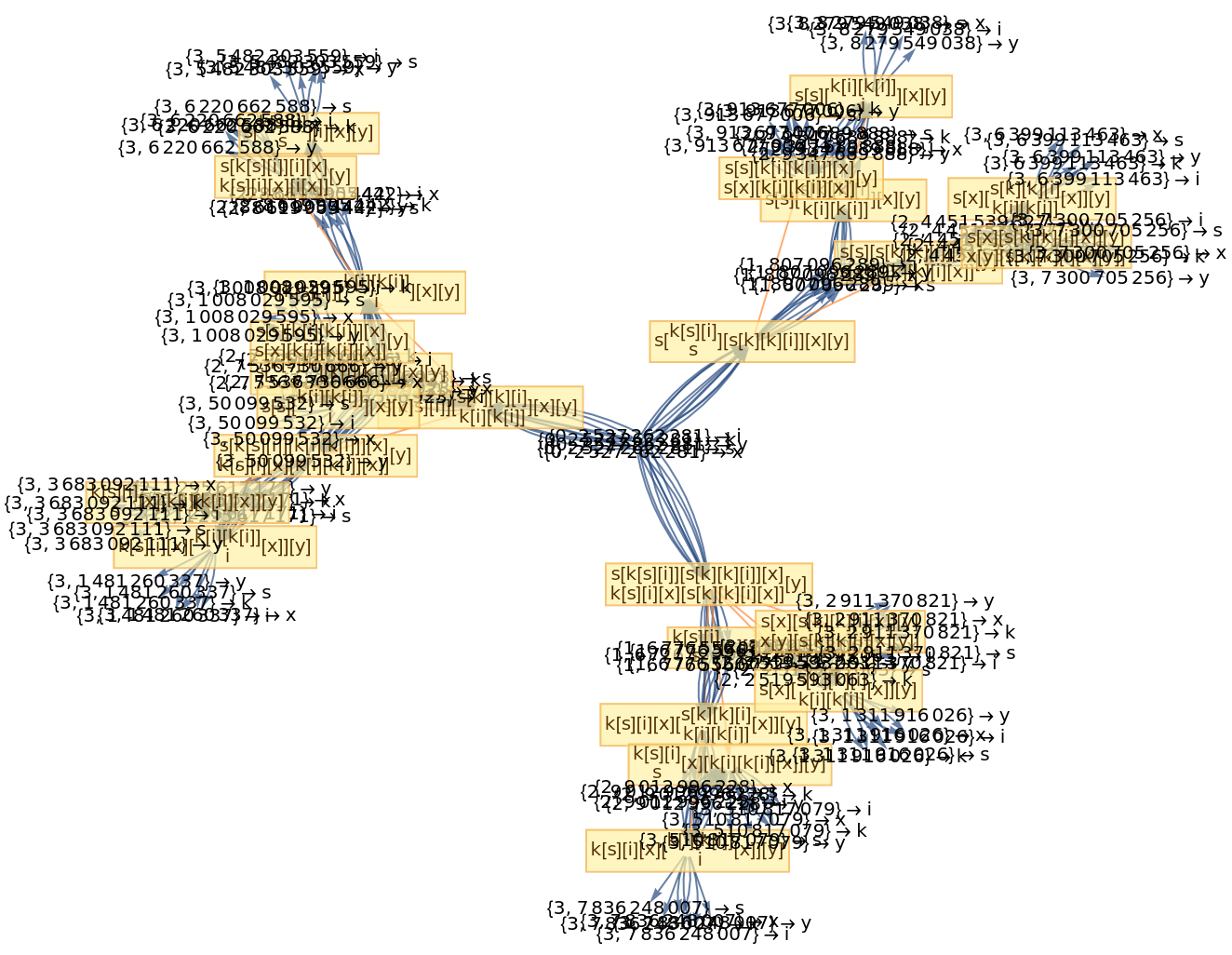Use a named shape as each state/token label:

 In:=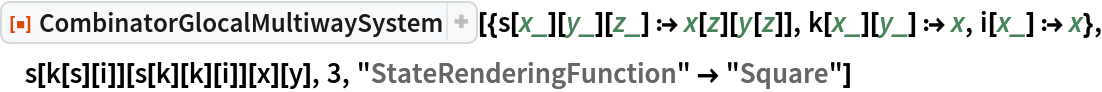Out=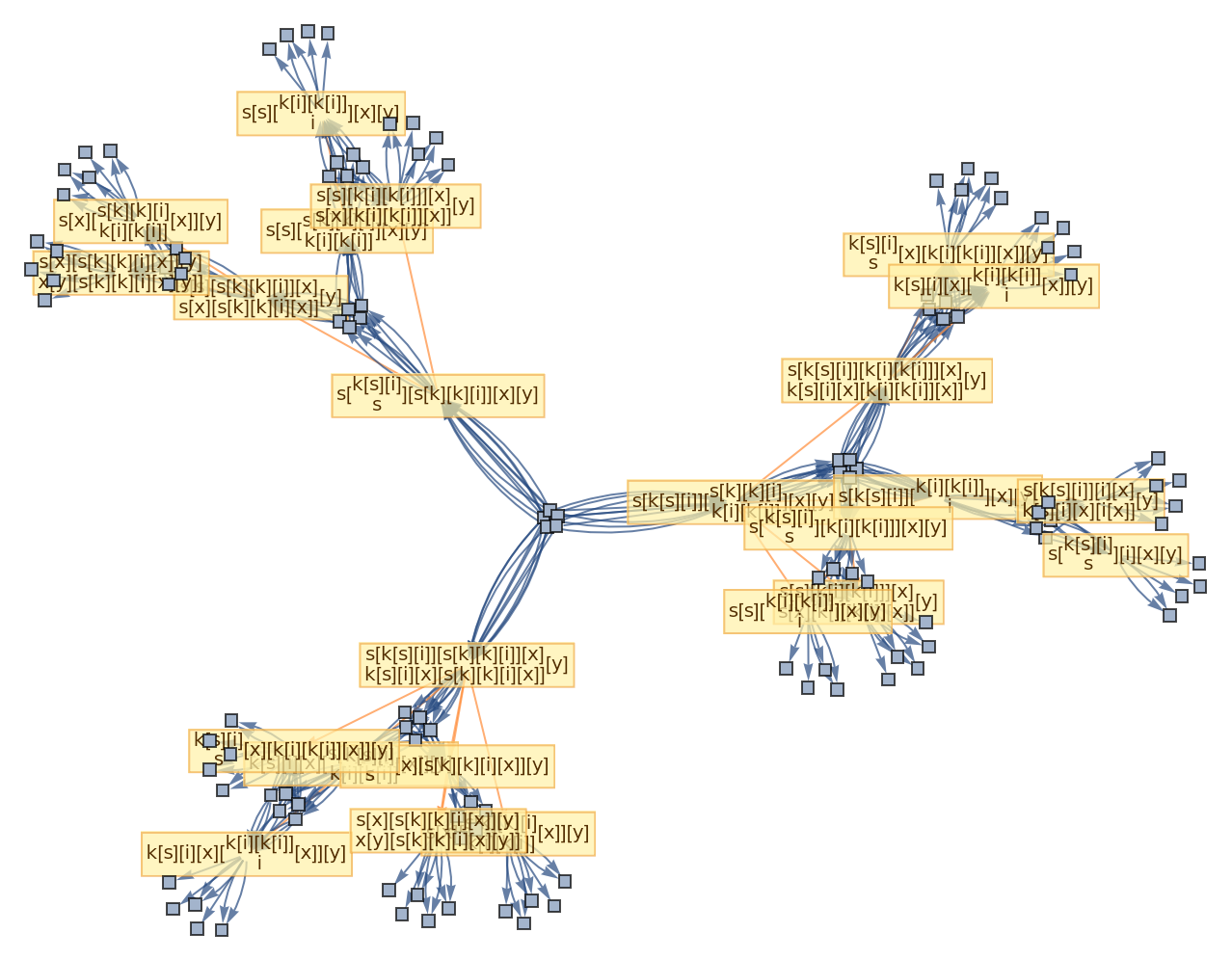#### Event vertex rendering (5)

By default, both states/tokens and events are labeled by their contents:

 In:=Out=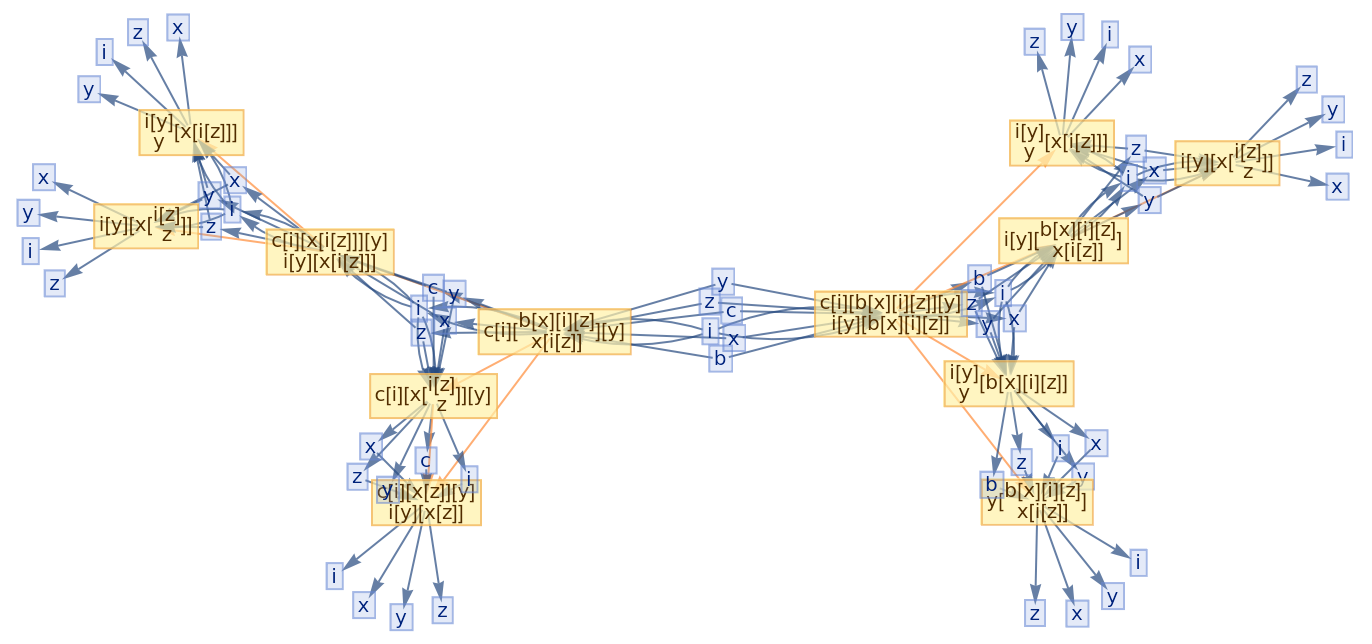Use no labeling for states/tokens:

 In:=Out=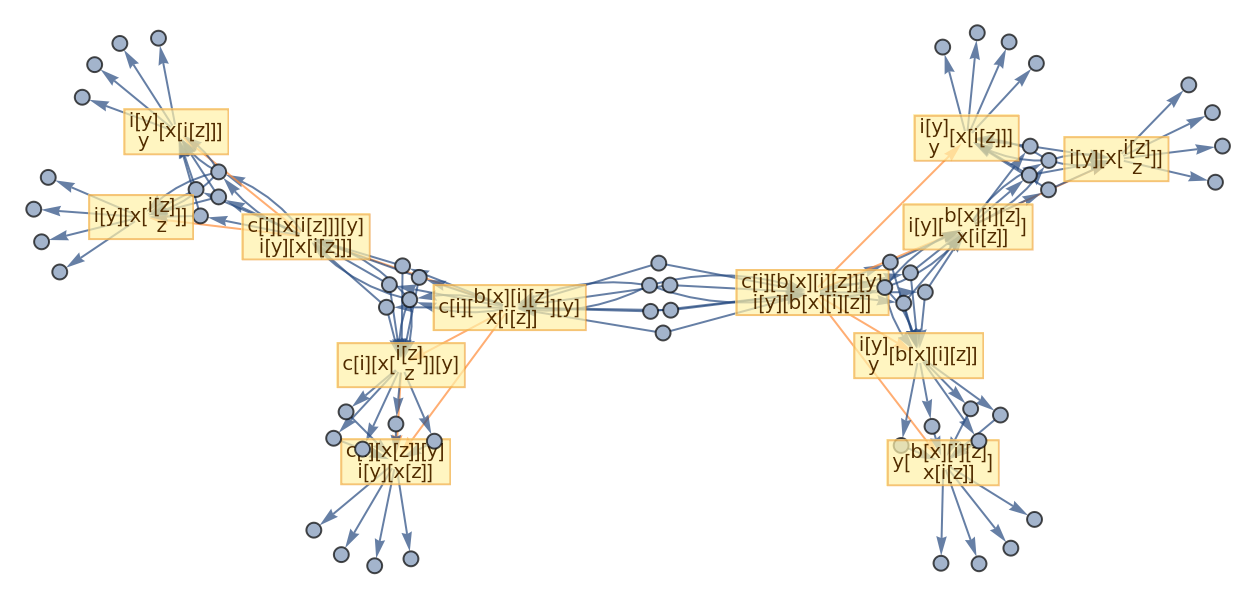Also use no labeling for events:

 In:=Out=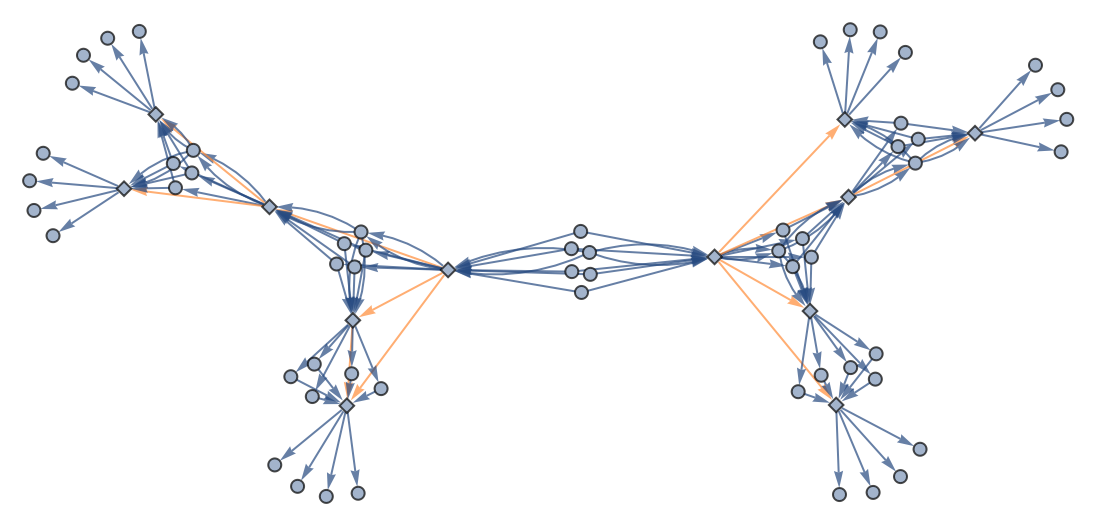Disabling vertex rendering yields an equivalent result:

 In:=Out=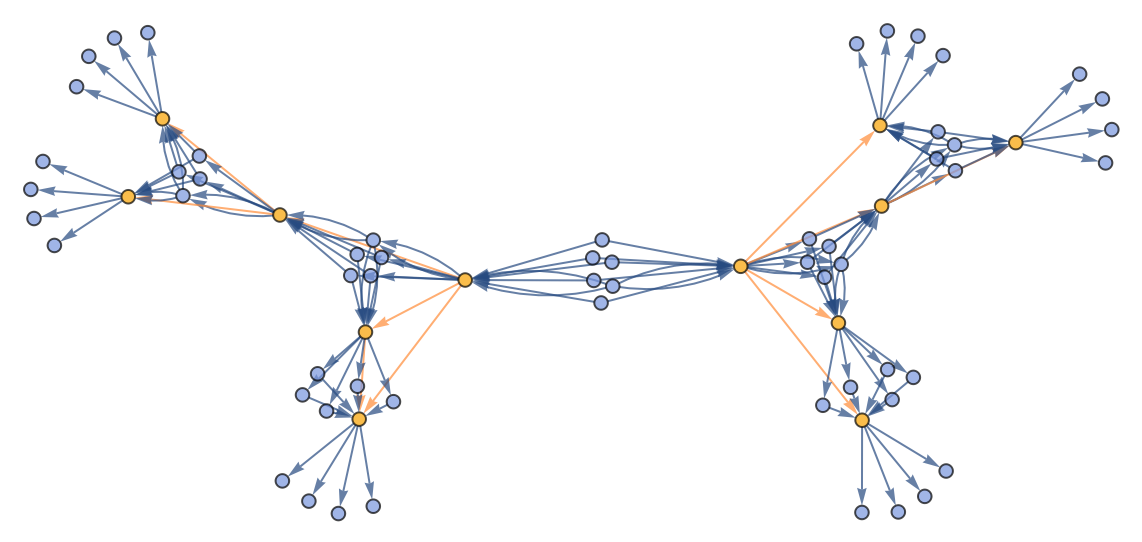Use raw event expressions as their labels:

 In:=Out=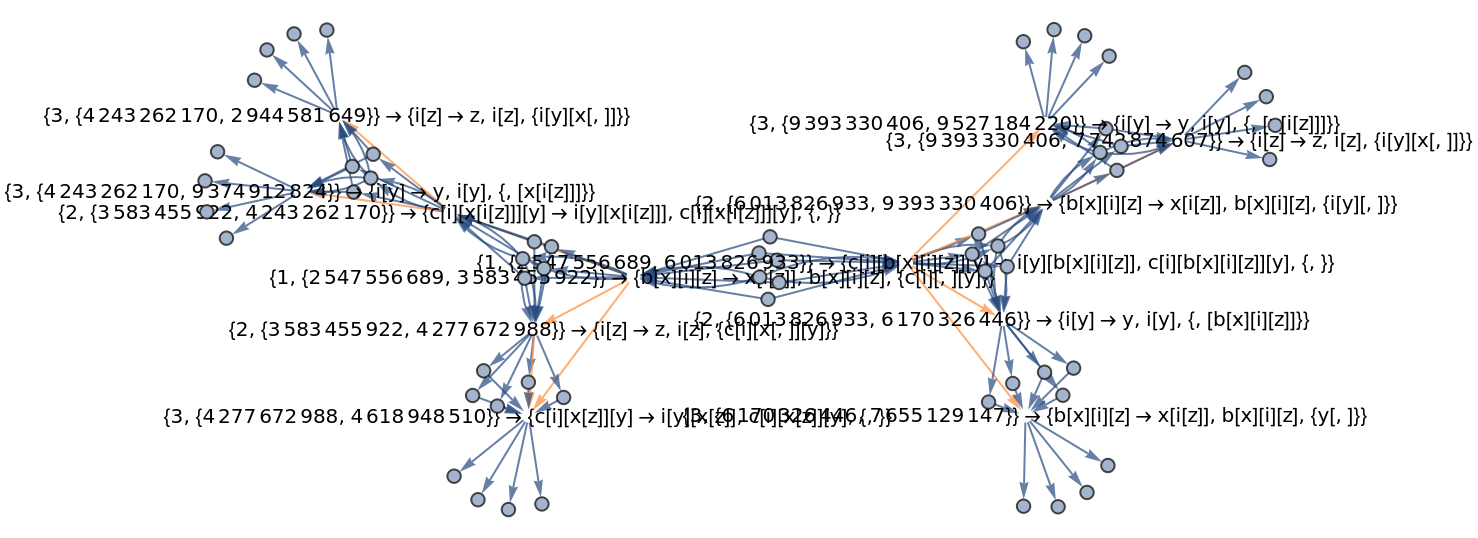#### Initialization events (3)

By default, combinator glocal multiway systems do not include initialization events:

 In:=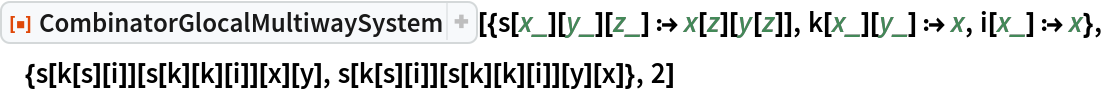Out=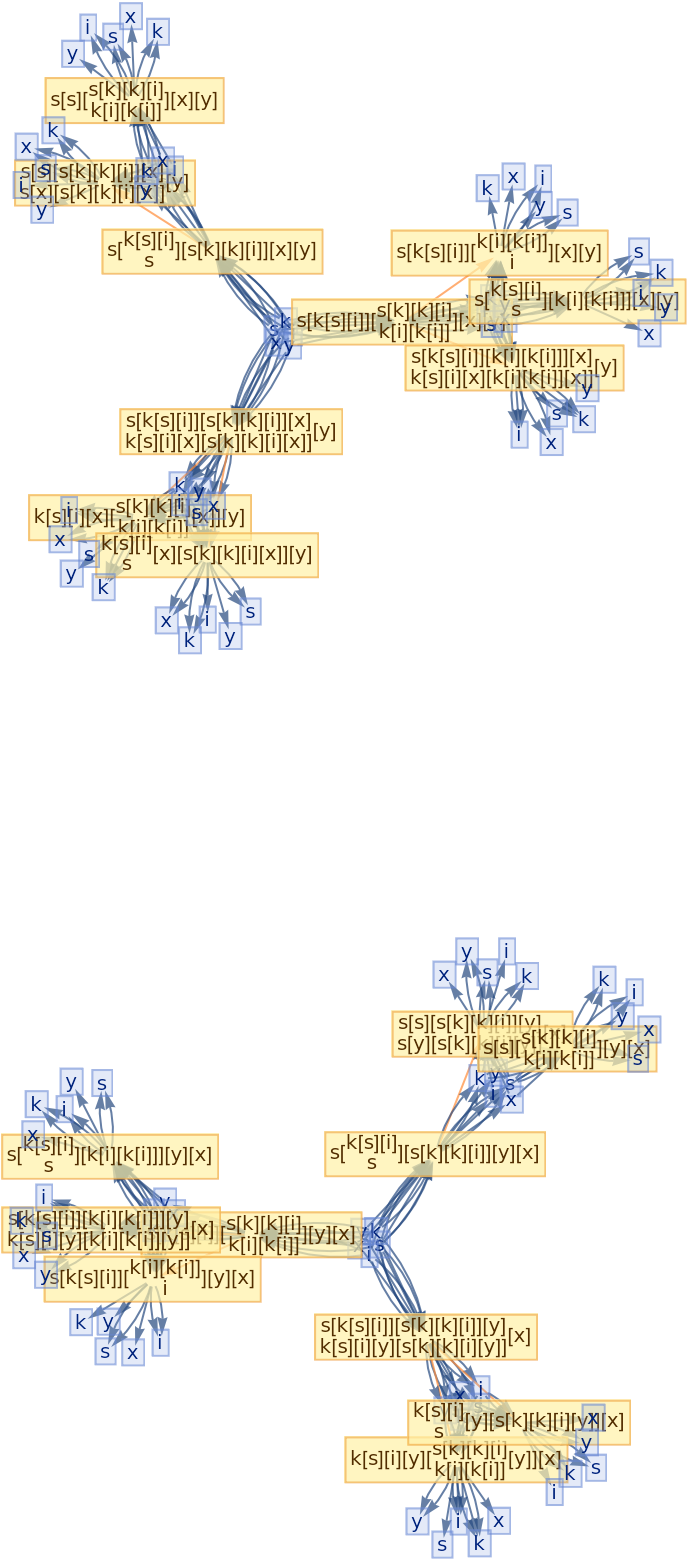The option "IncludeInitializationEvents" allows one to override this default (note that initialization events have special default rendering):

 In:=Out=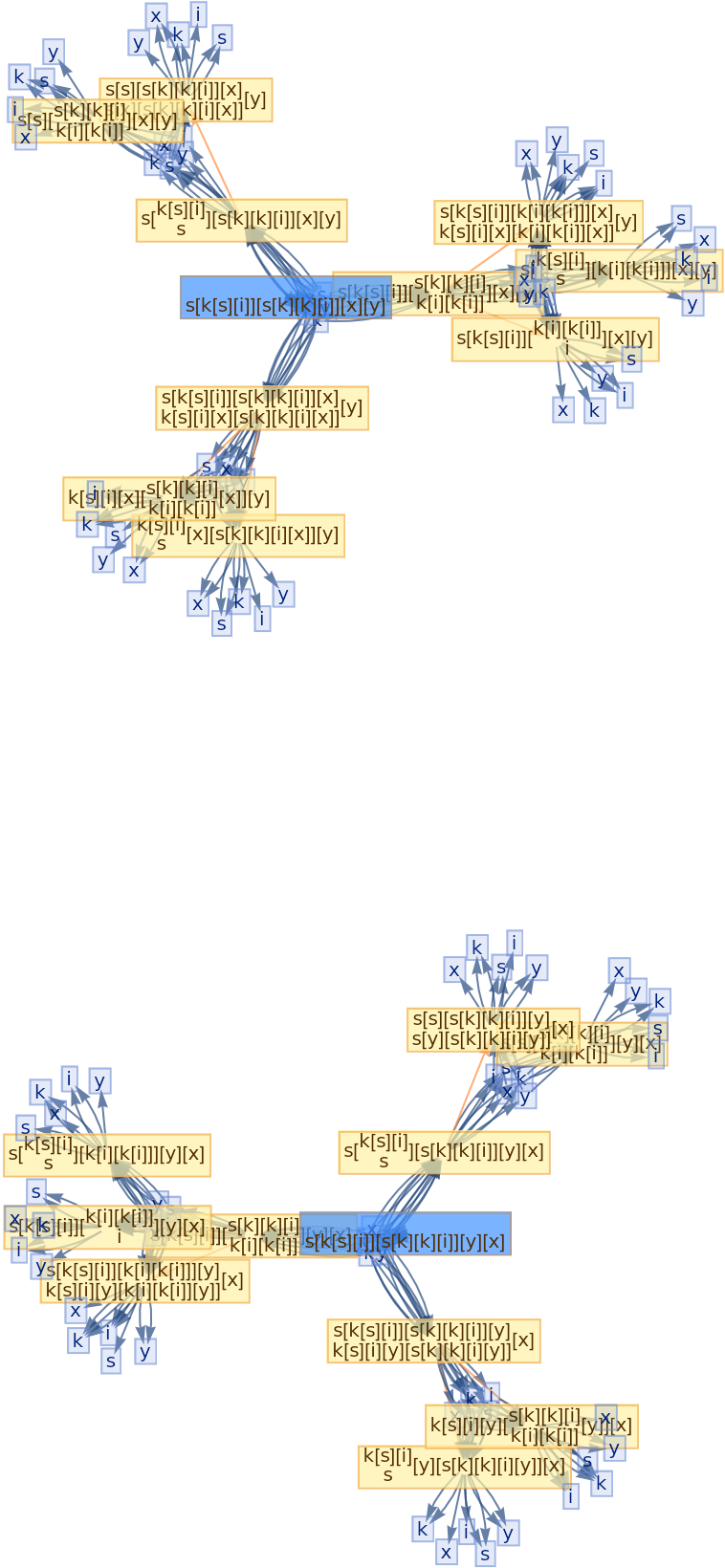Disable vertex rendering:

 In:=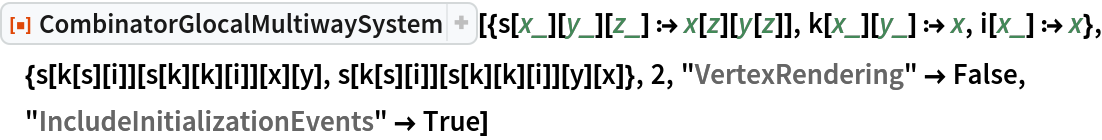Out=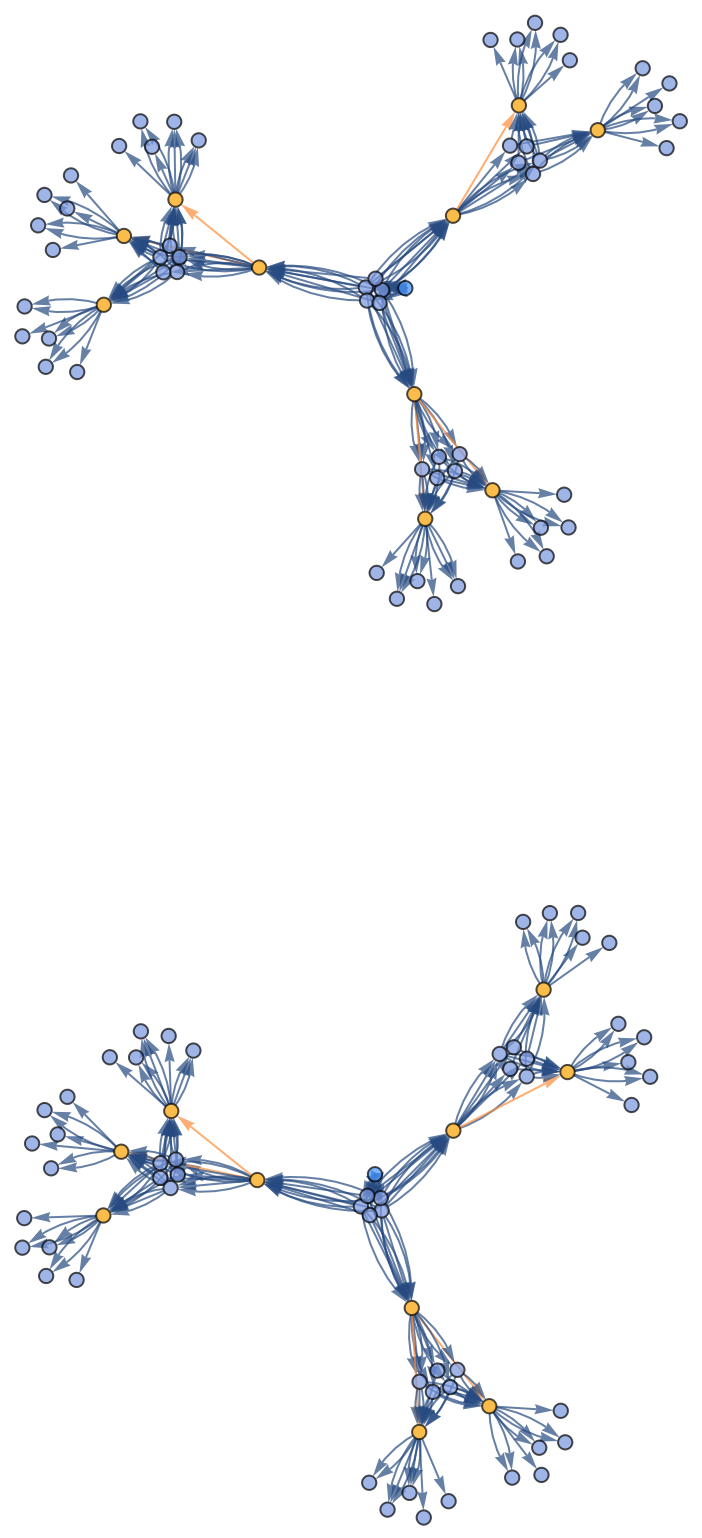#### Graph layout options (3)

Place arrows in the middle of edges:

 In:=Out=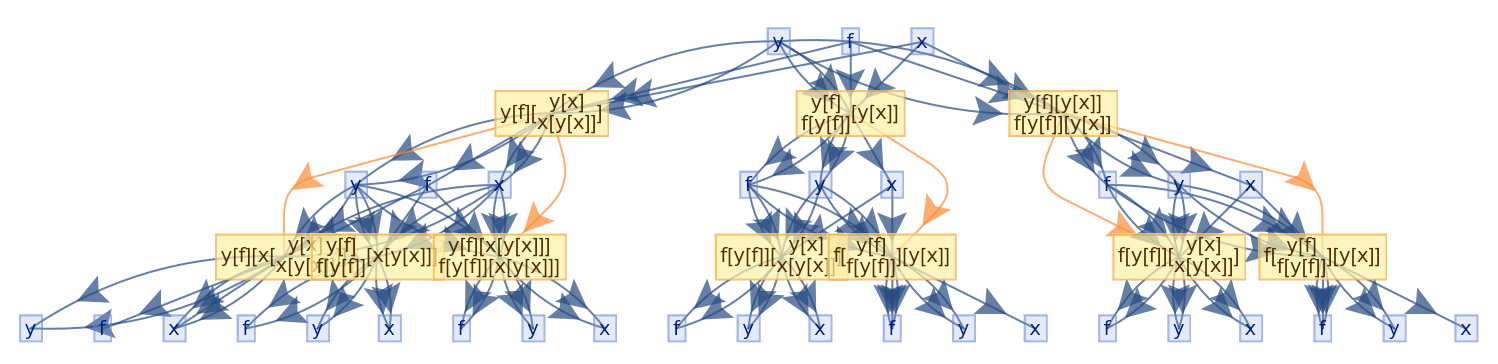Generate an example of glocal multiway combinator evaluation:

 In:=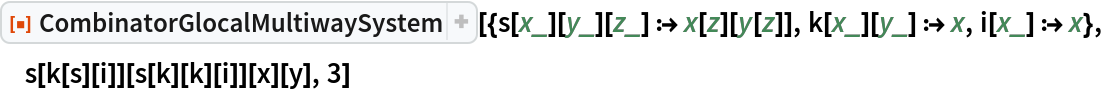Out=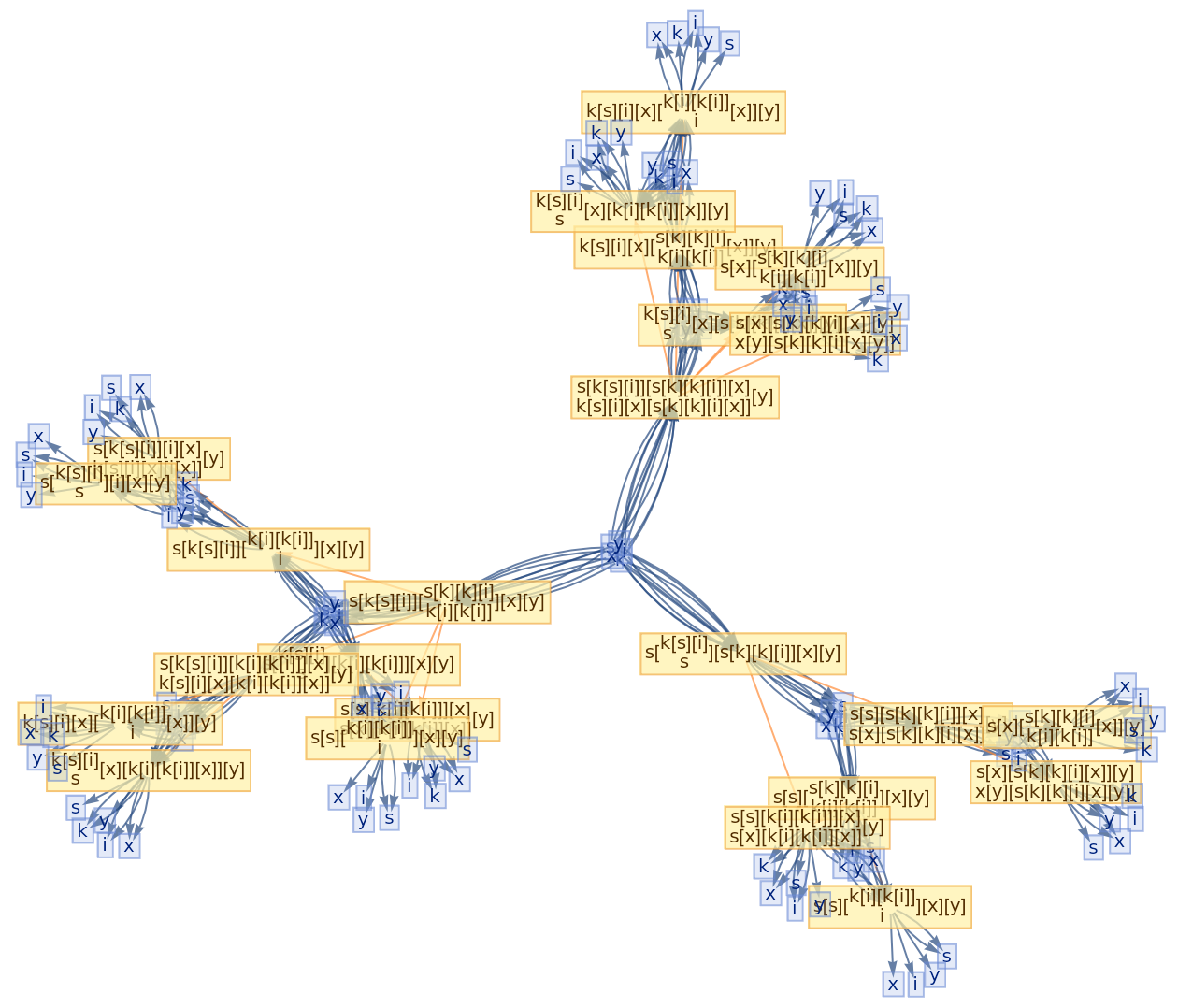Force a layered digraph embedding (with the initial state/tokens at the top):

 In:=Out=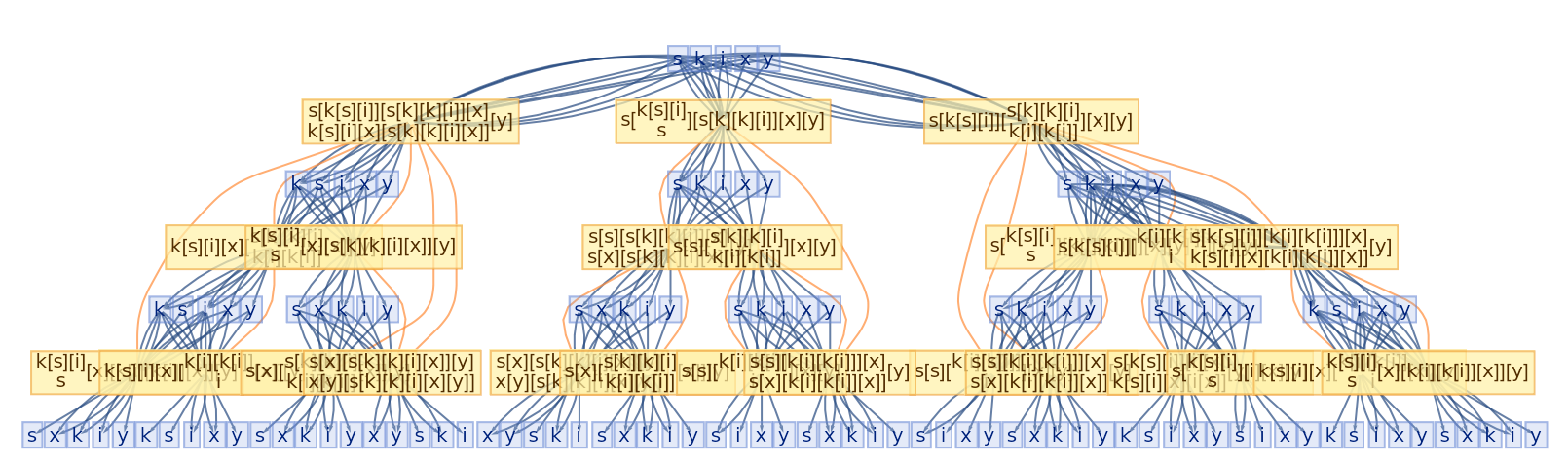Jonathan Gorard

## Version History

• 1.0.0 – 03 January 2022